# ICSE Class 10 Chemistry Question Paper Solution 2016

ICSE Class 10 Chemistry 2016 question paper contains questions from Organic Chemistry, Inorganic Chemistry, Physical Chemistry and Biochemistry. Solving the 2016 Question Paper and referring to the ICSE Class 10 Chemistry Question Paper 2016 Solution will help students in understanding the important topics from where questions are asked in the exam. Also, they will know the changes that have been made in the paper pattern from 2016 to the present and accordingly they can prepare for the exam in a better way. Also, students get an idea on types of questions expected to be asked in the upcoming Chemistry exam.

The ICSE Class 10 Chemistry 2016 exam was conducted for 2 hours of time duration. The paper was of moderate difficulty level although few questions were difficult to solve. Here we have provided the complete ICSE Class 10 Chemistry Question Paper Solution 2016. Students can download the Solution PDF from the link below for future reference.

Students can have a look at the ICSE Class 10 Chemistry Question Paper Solution 2016 below: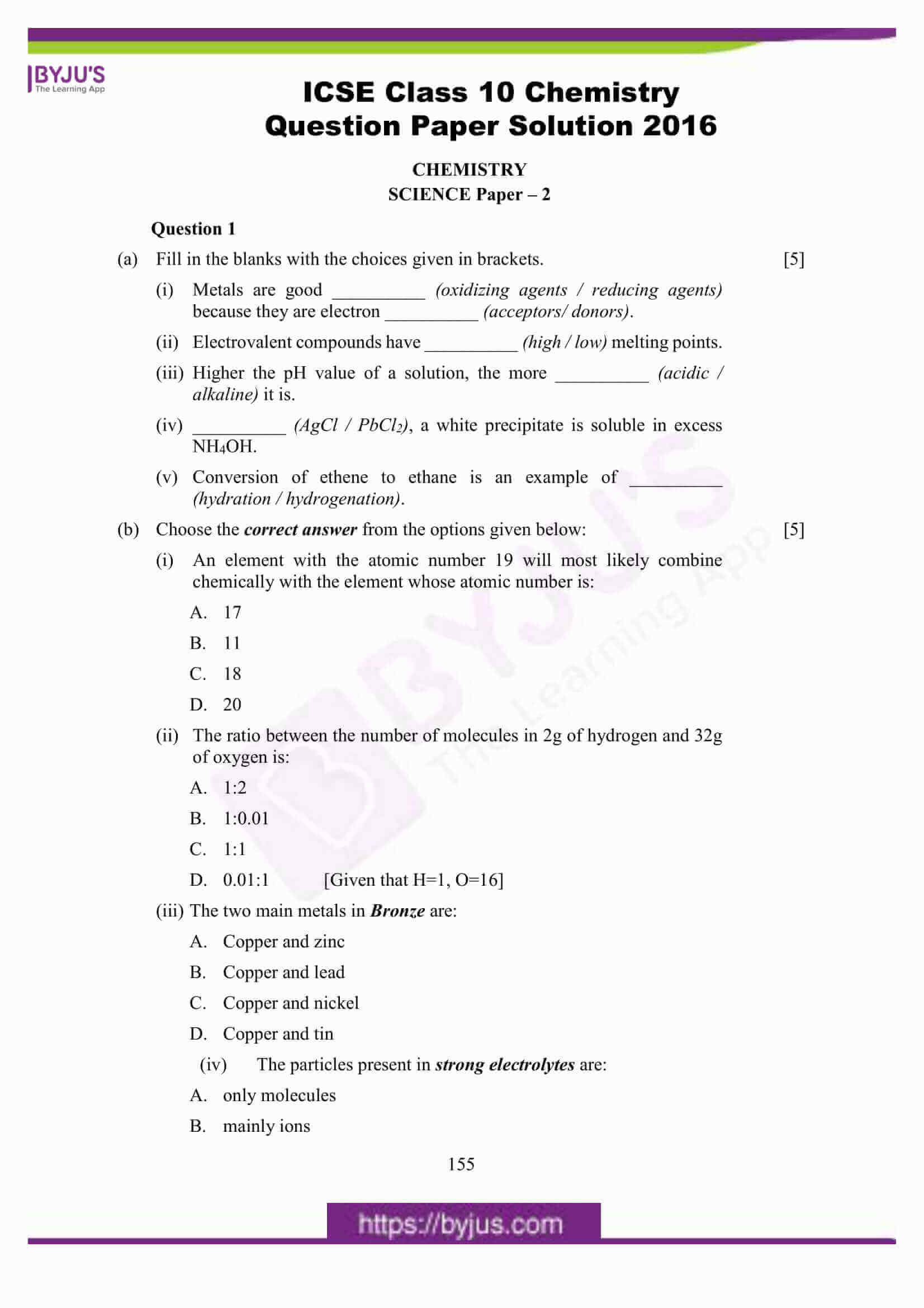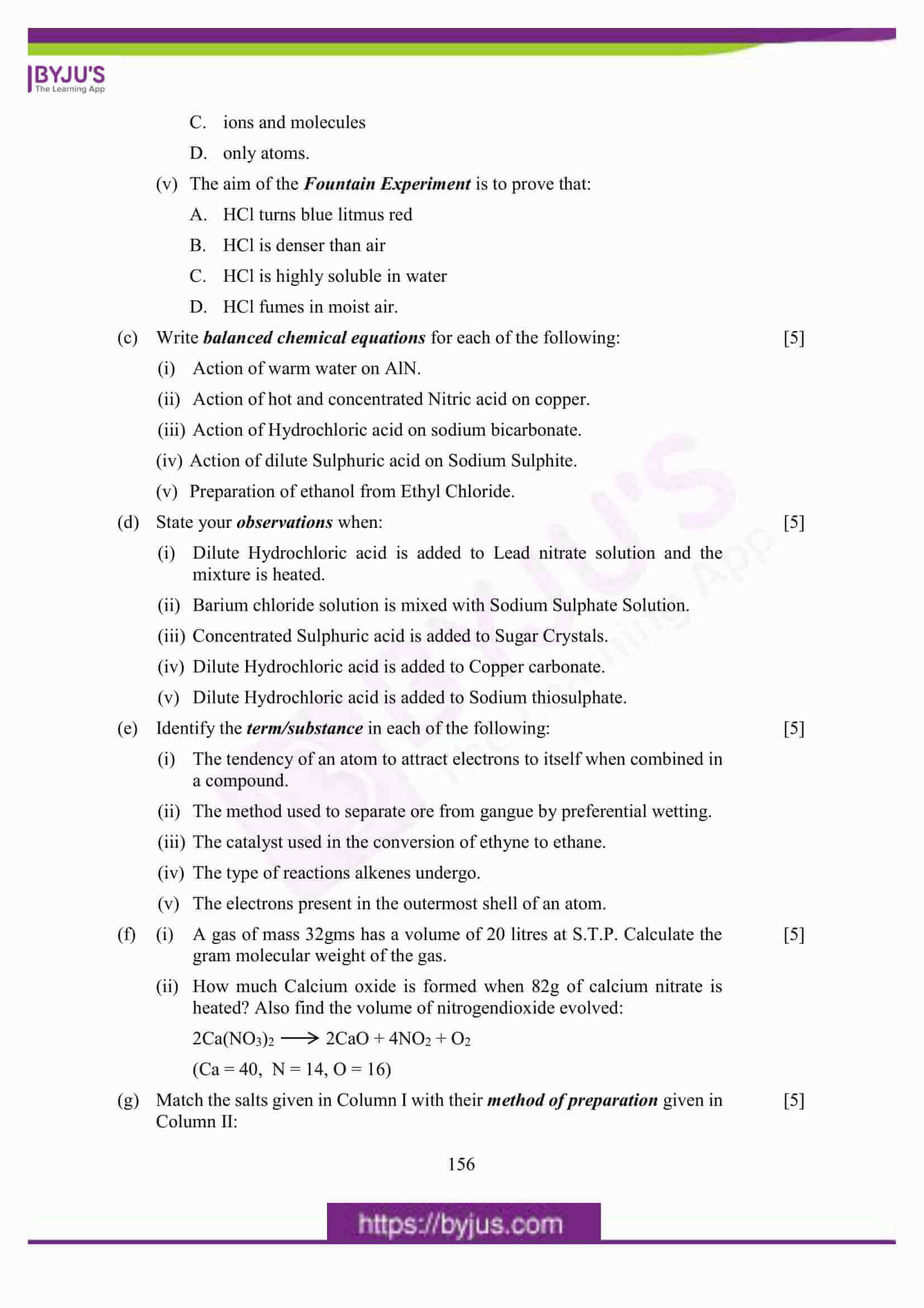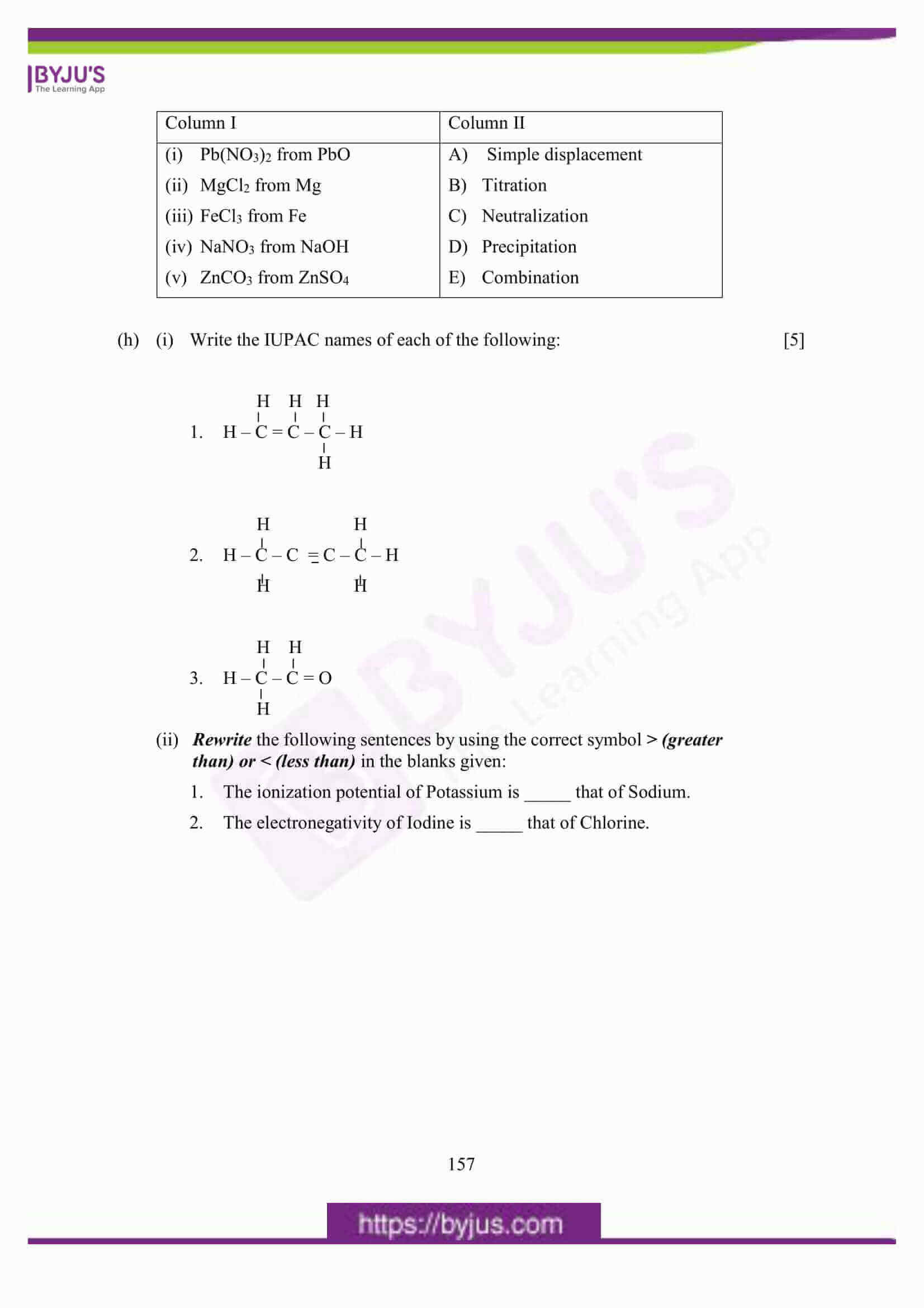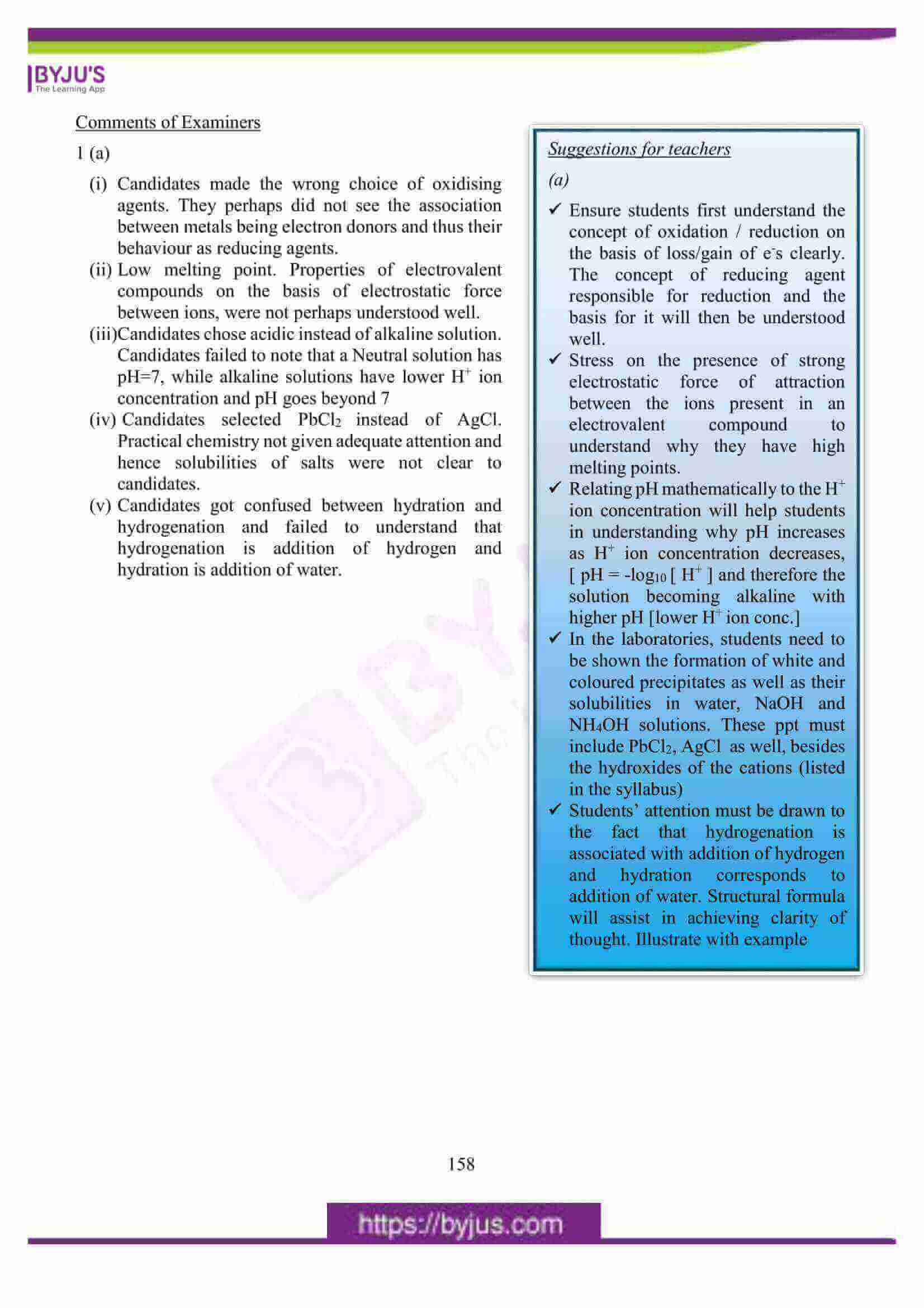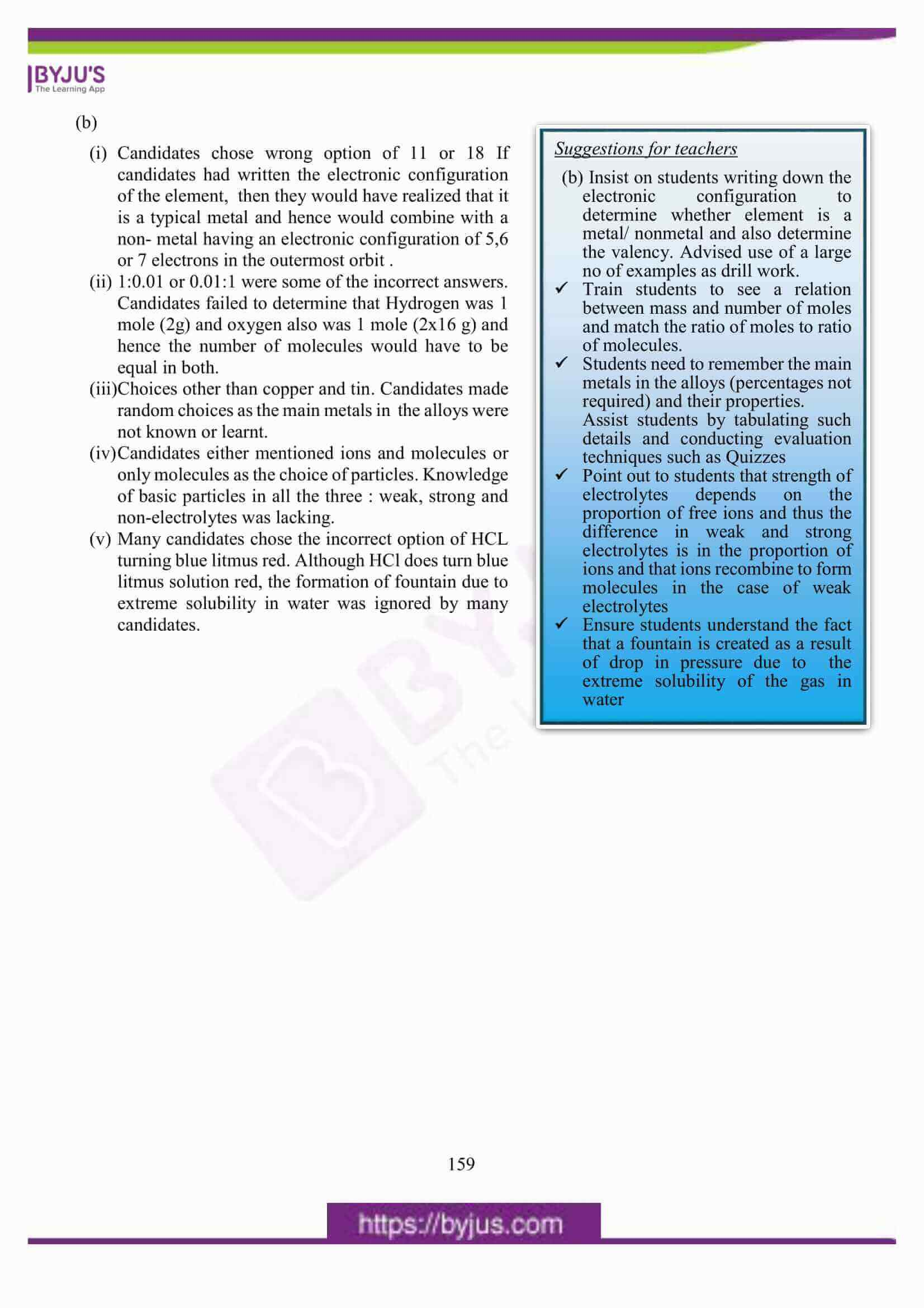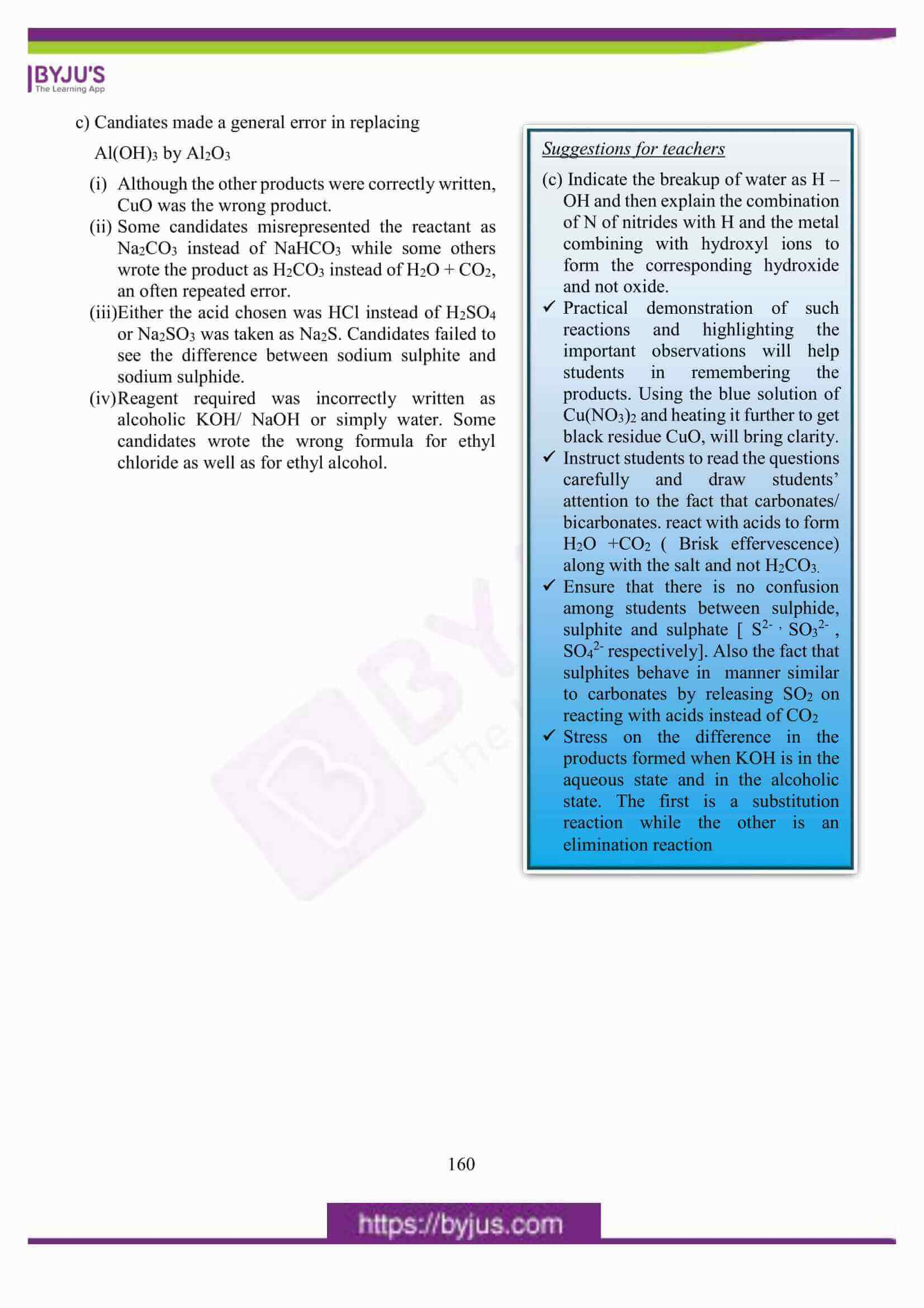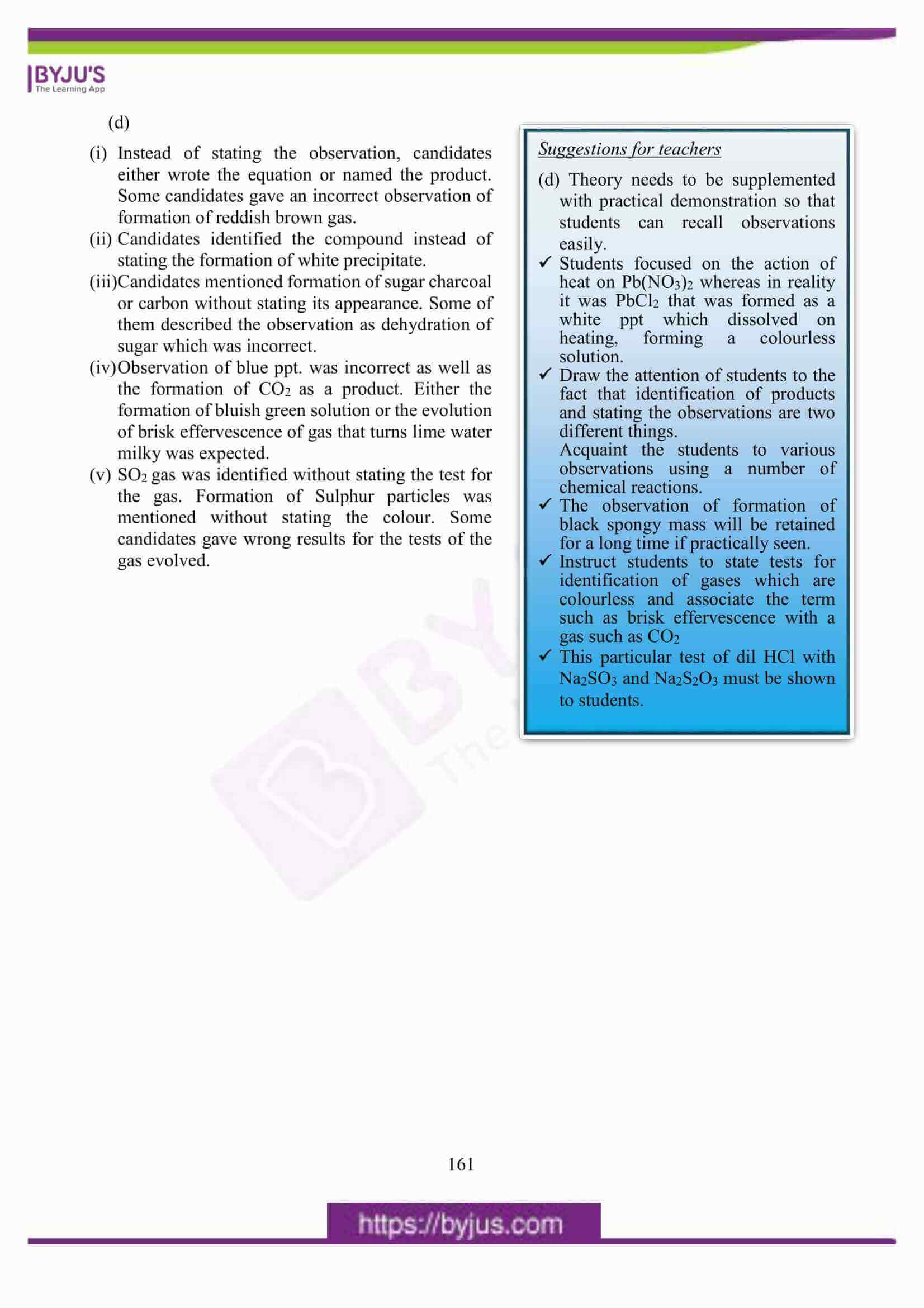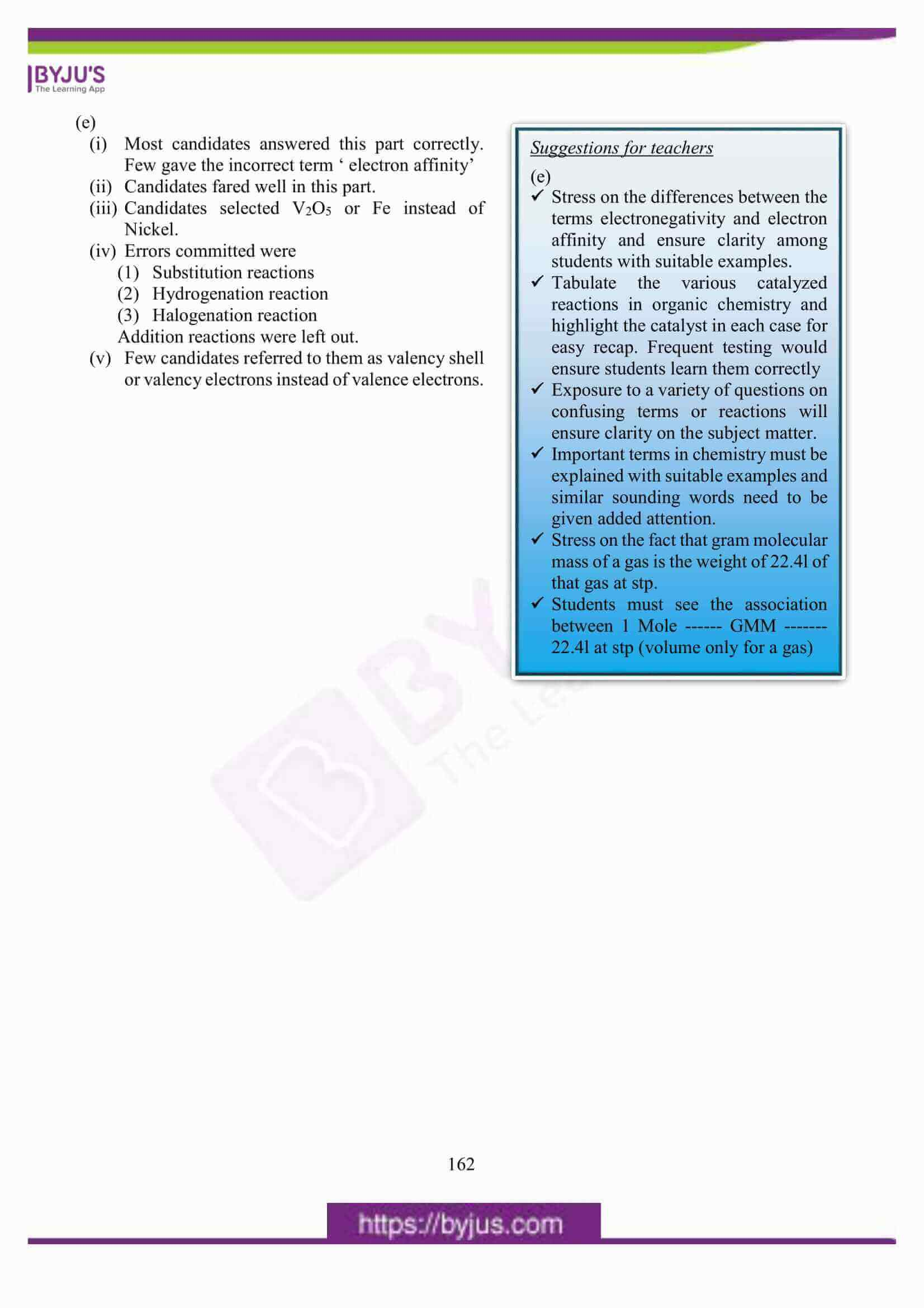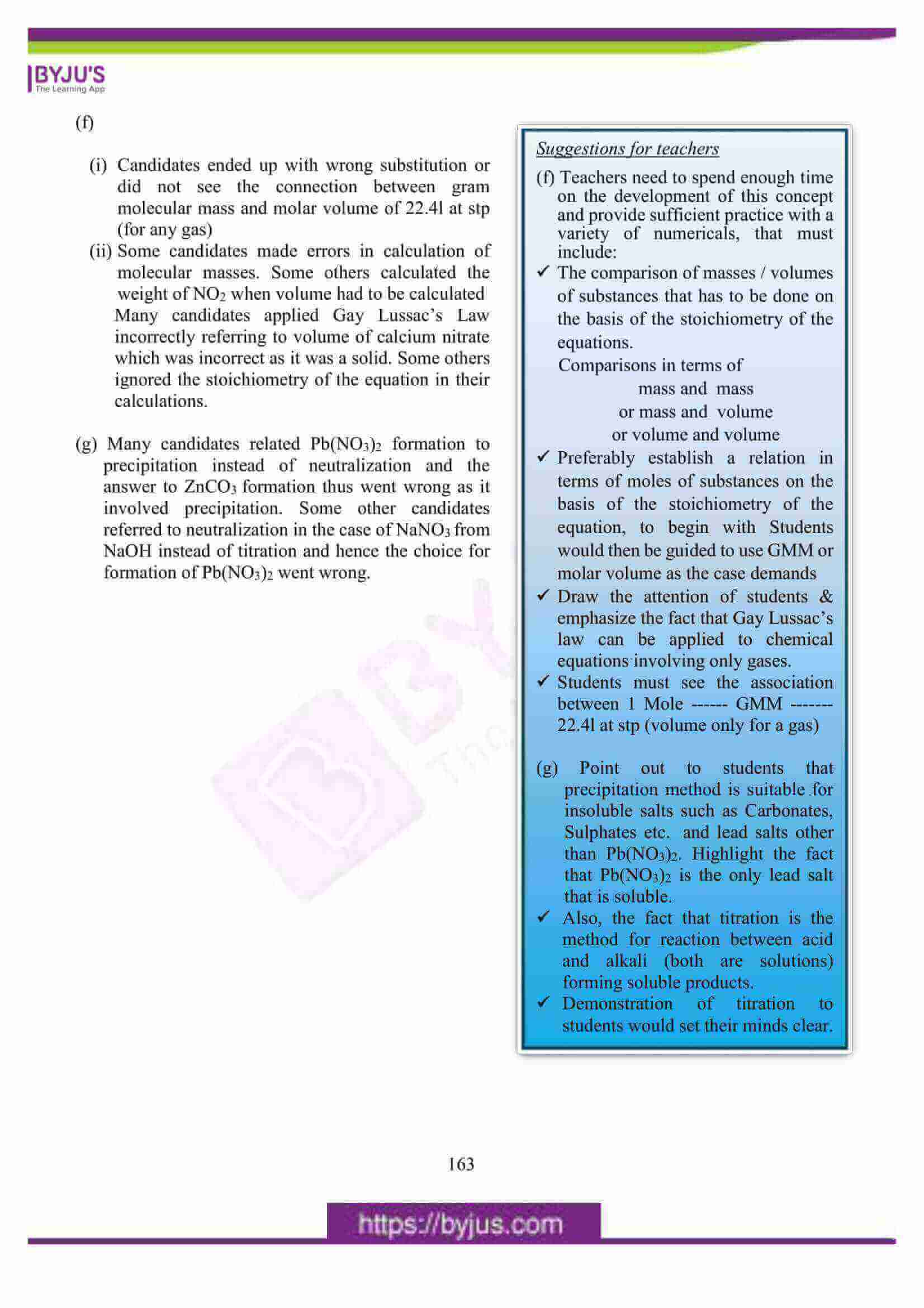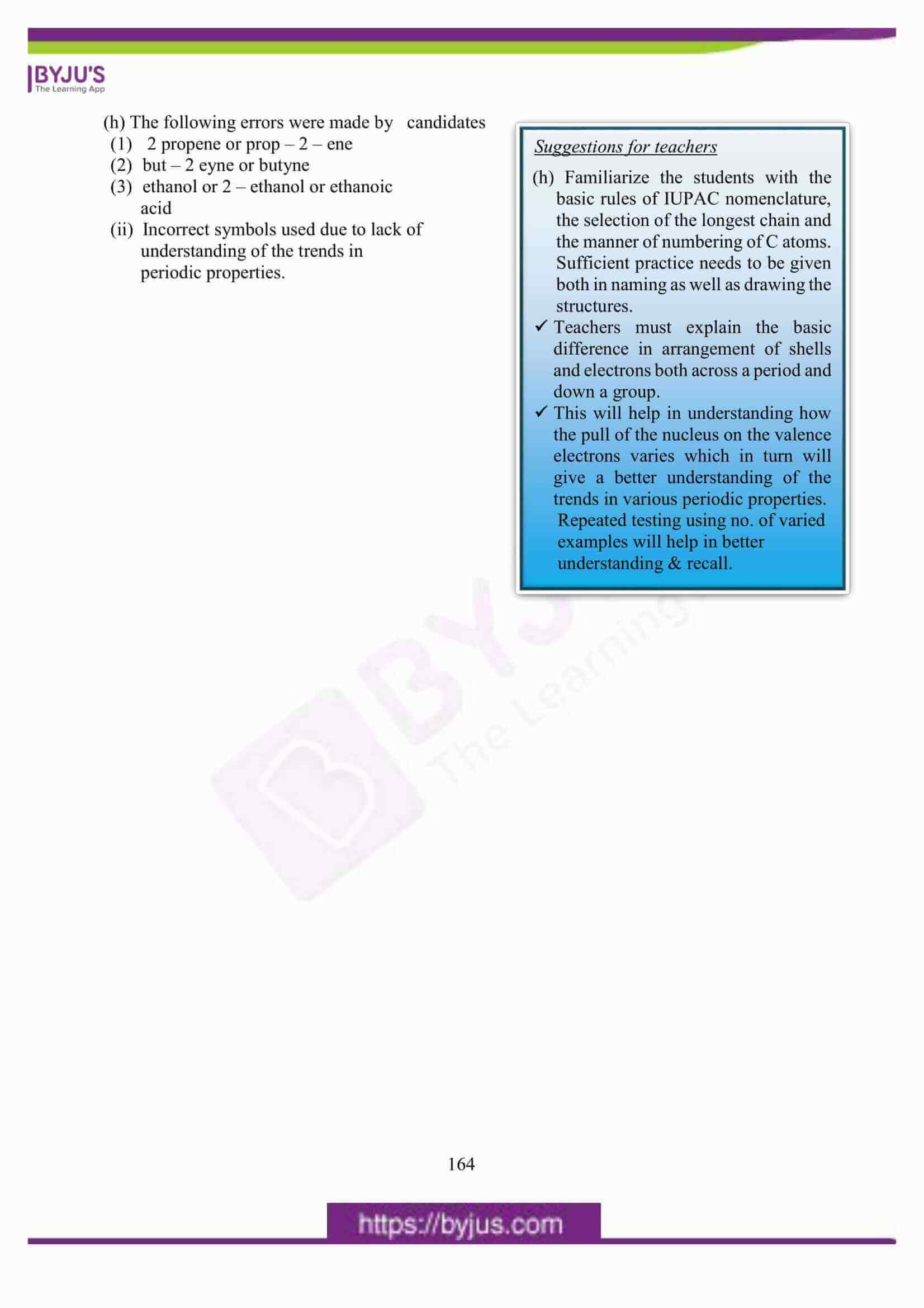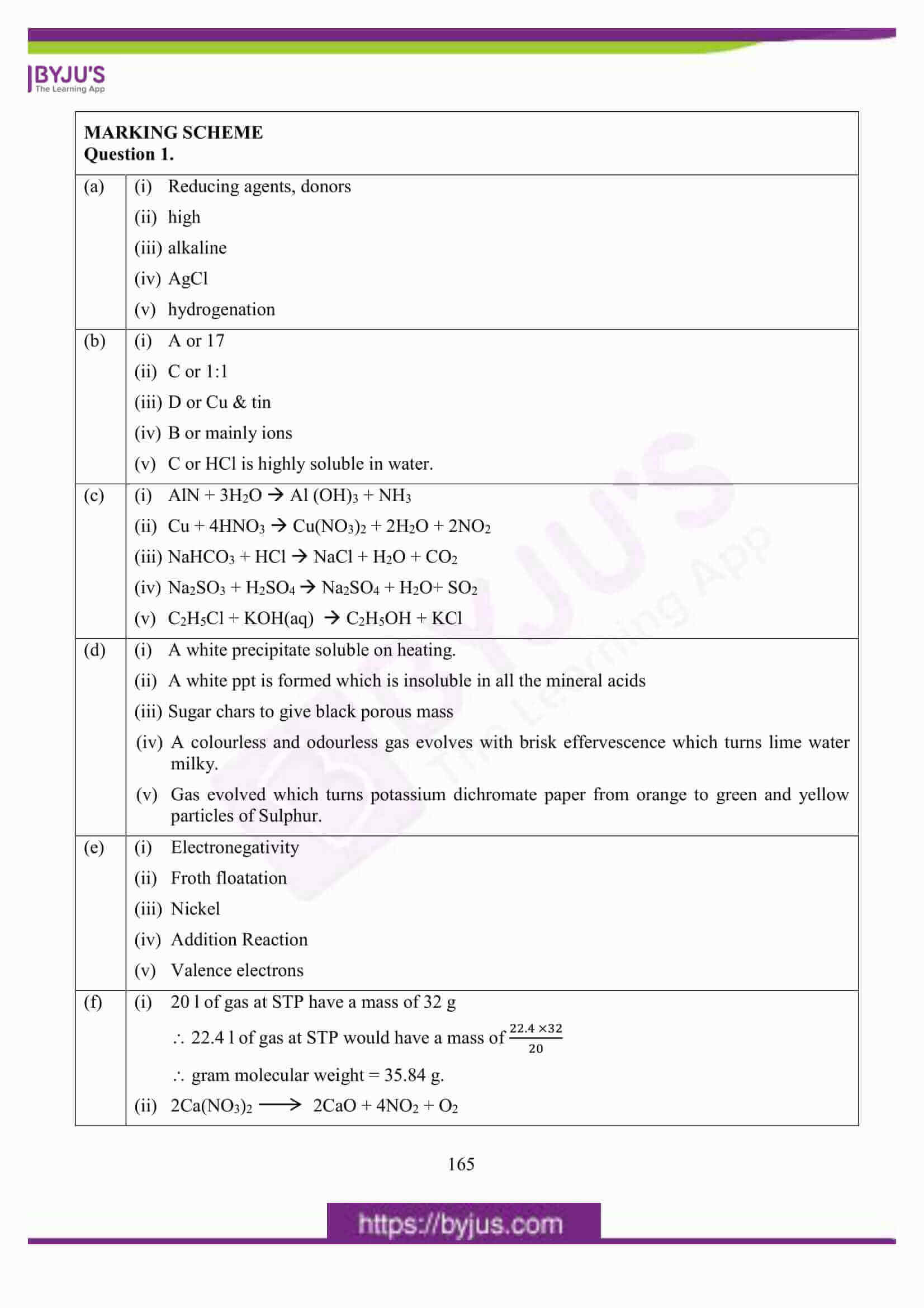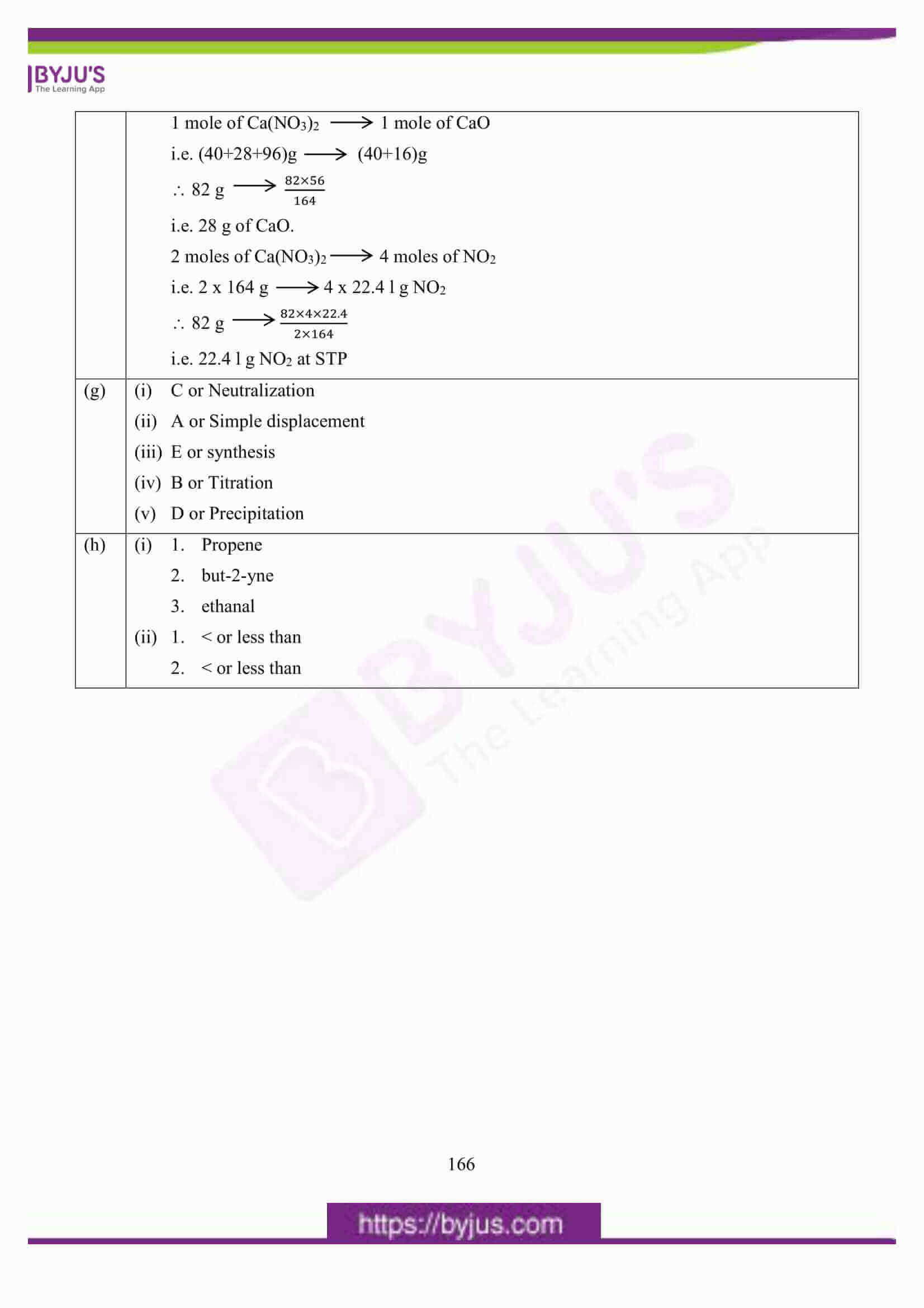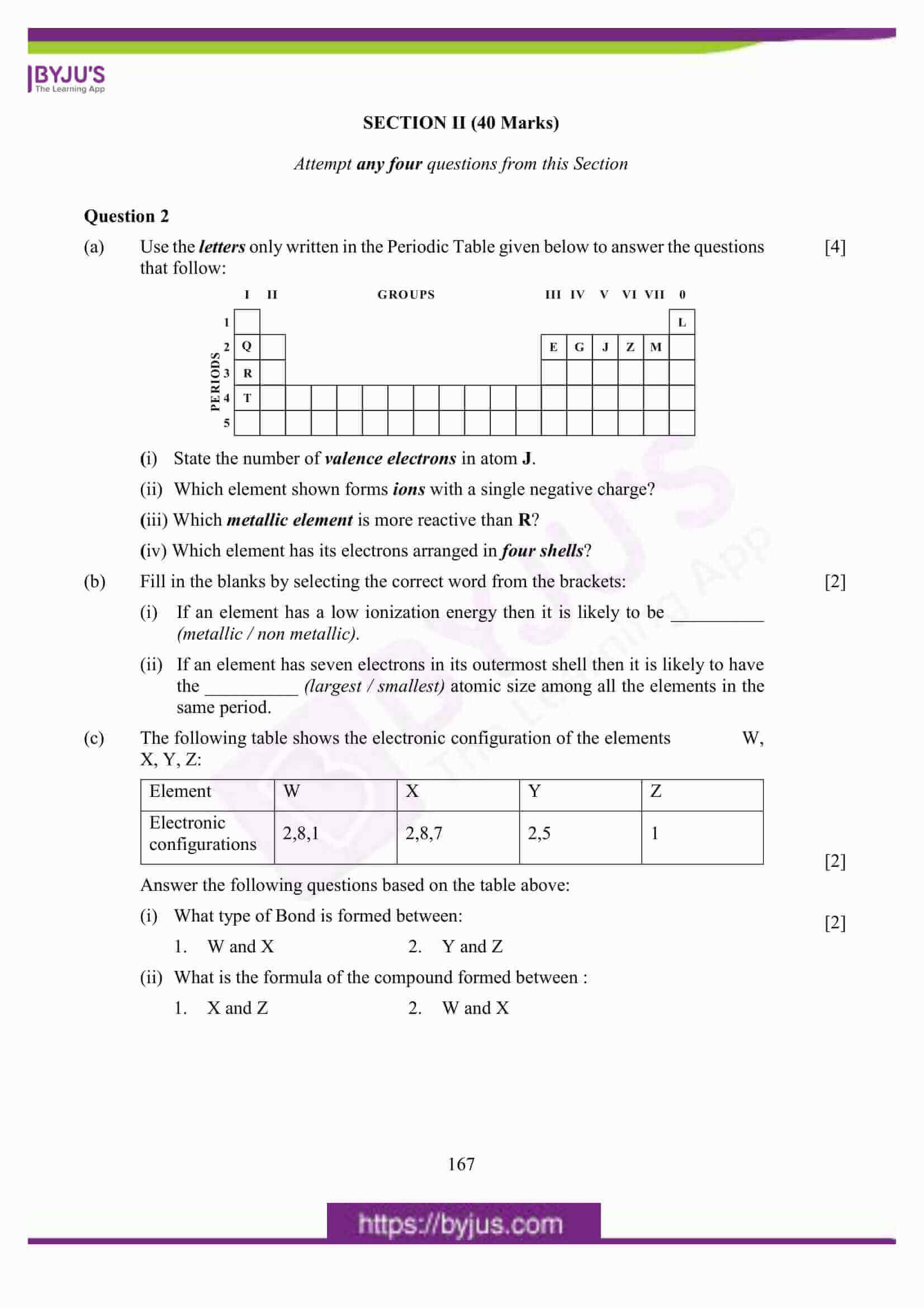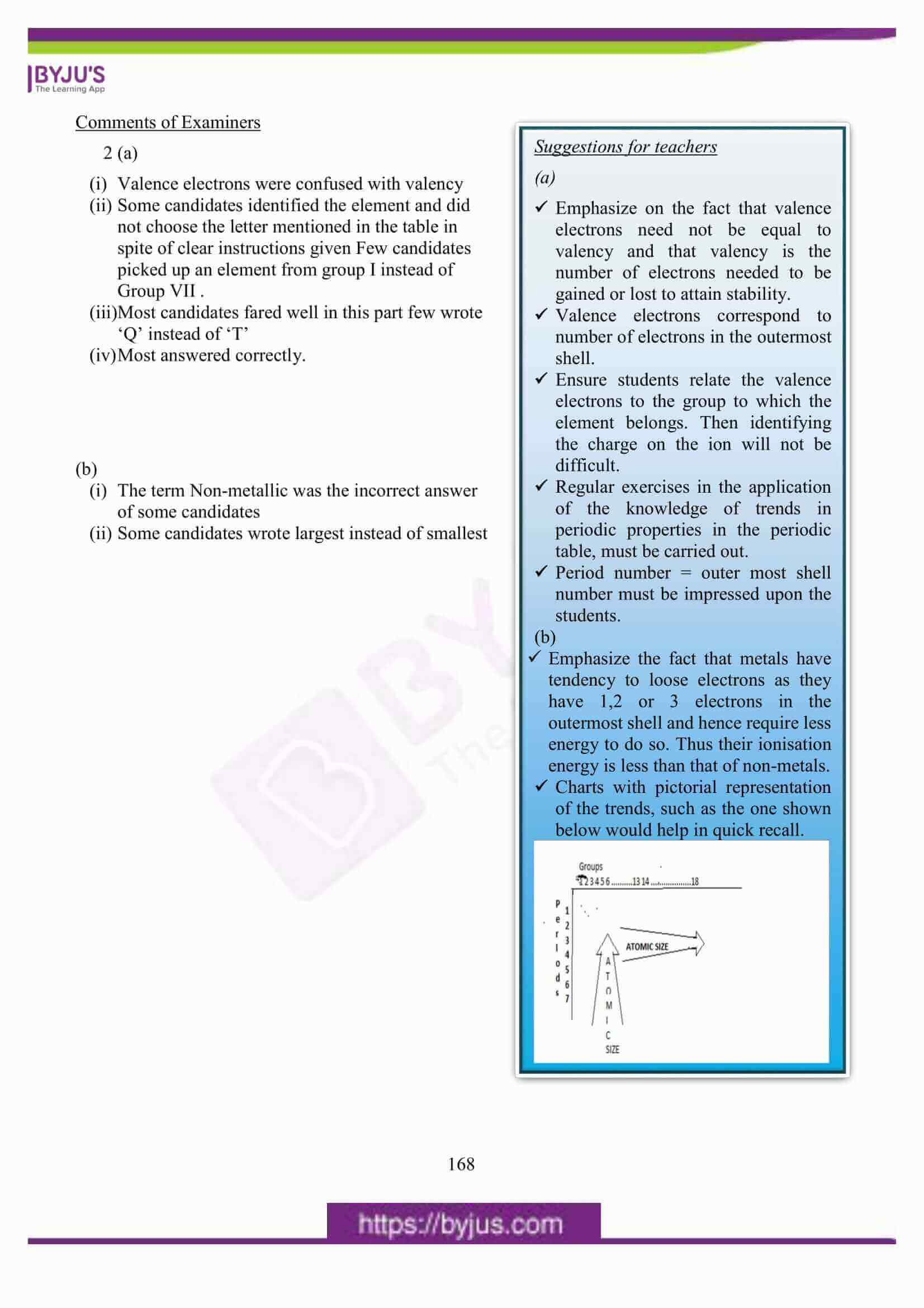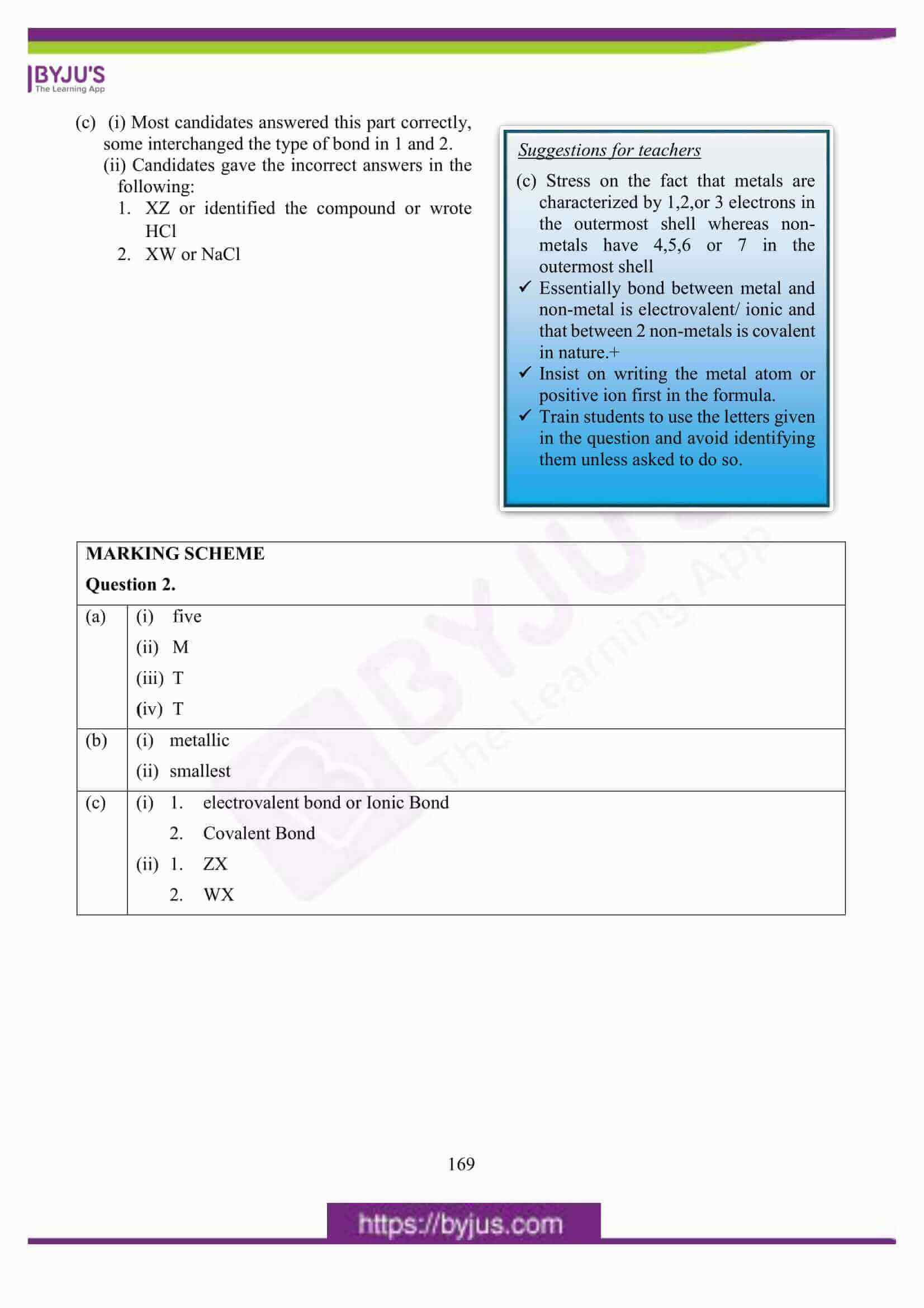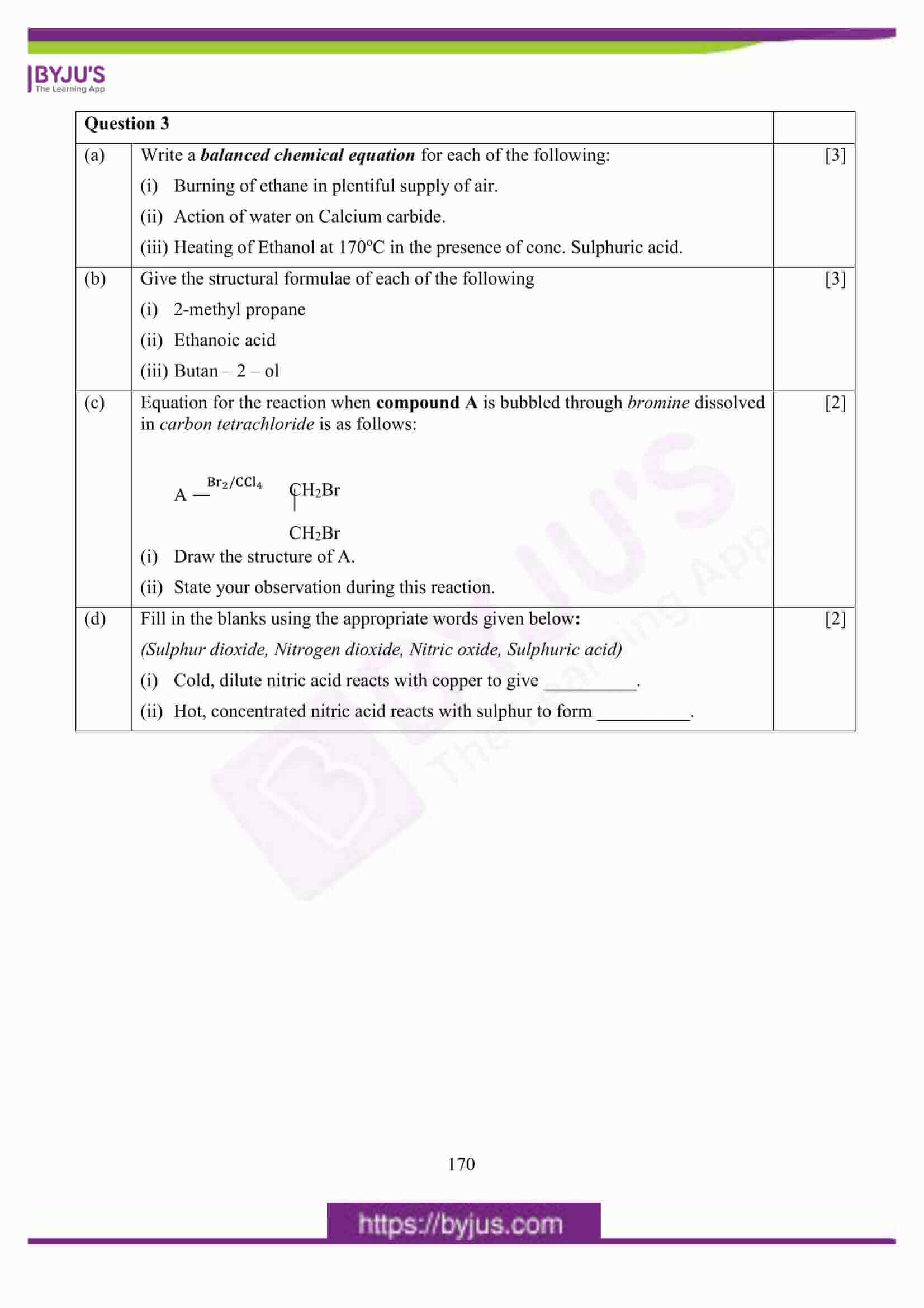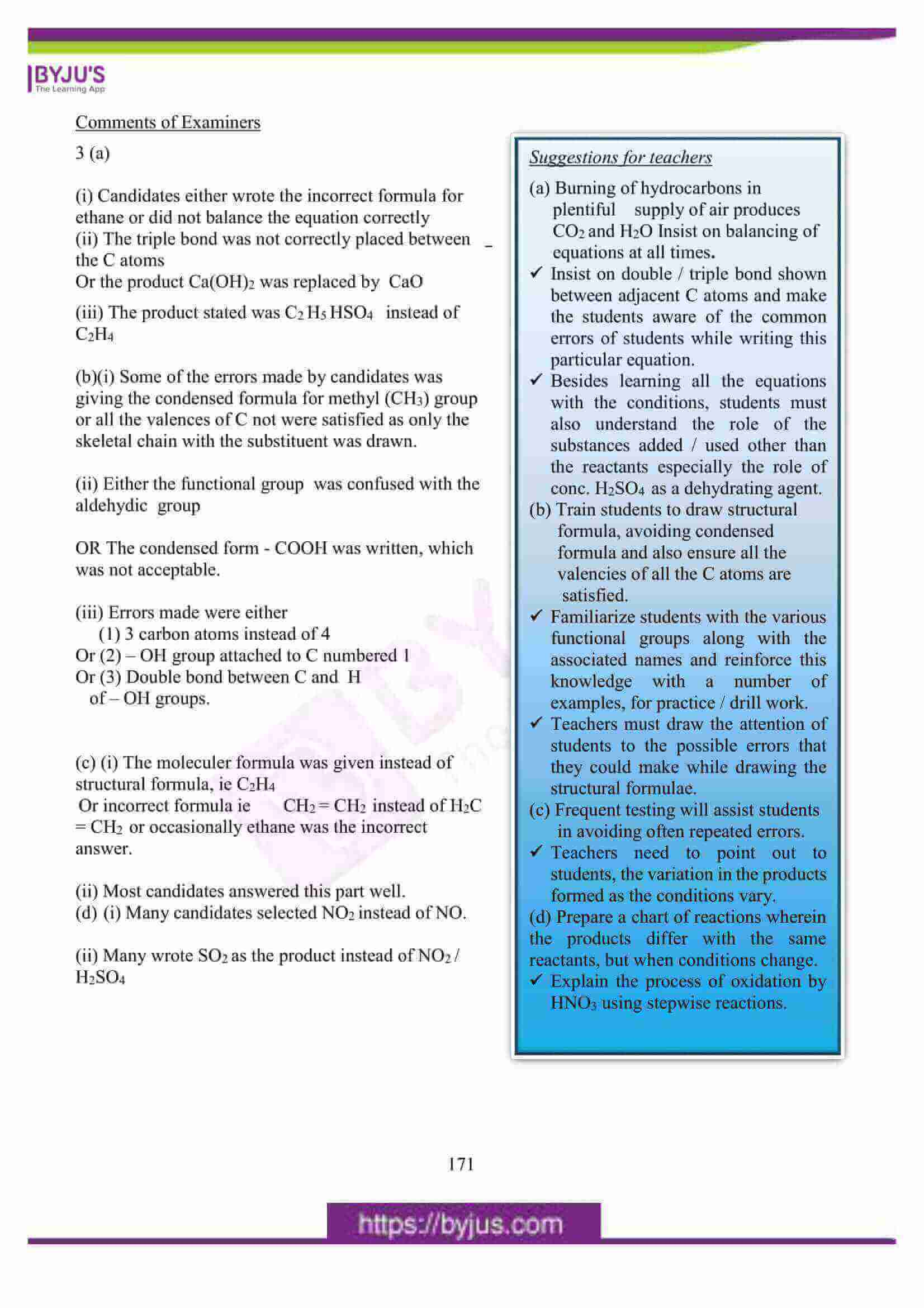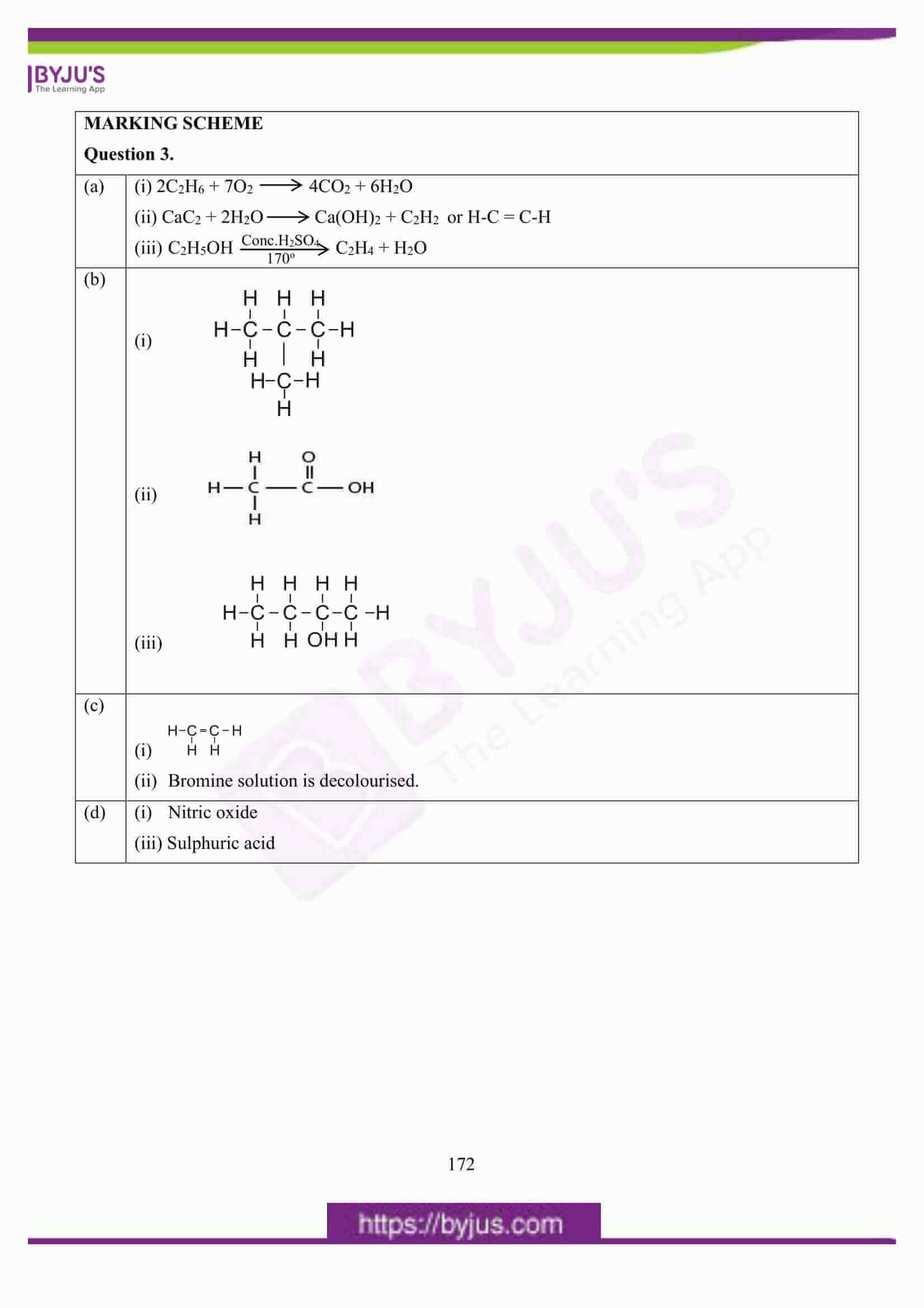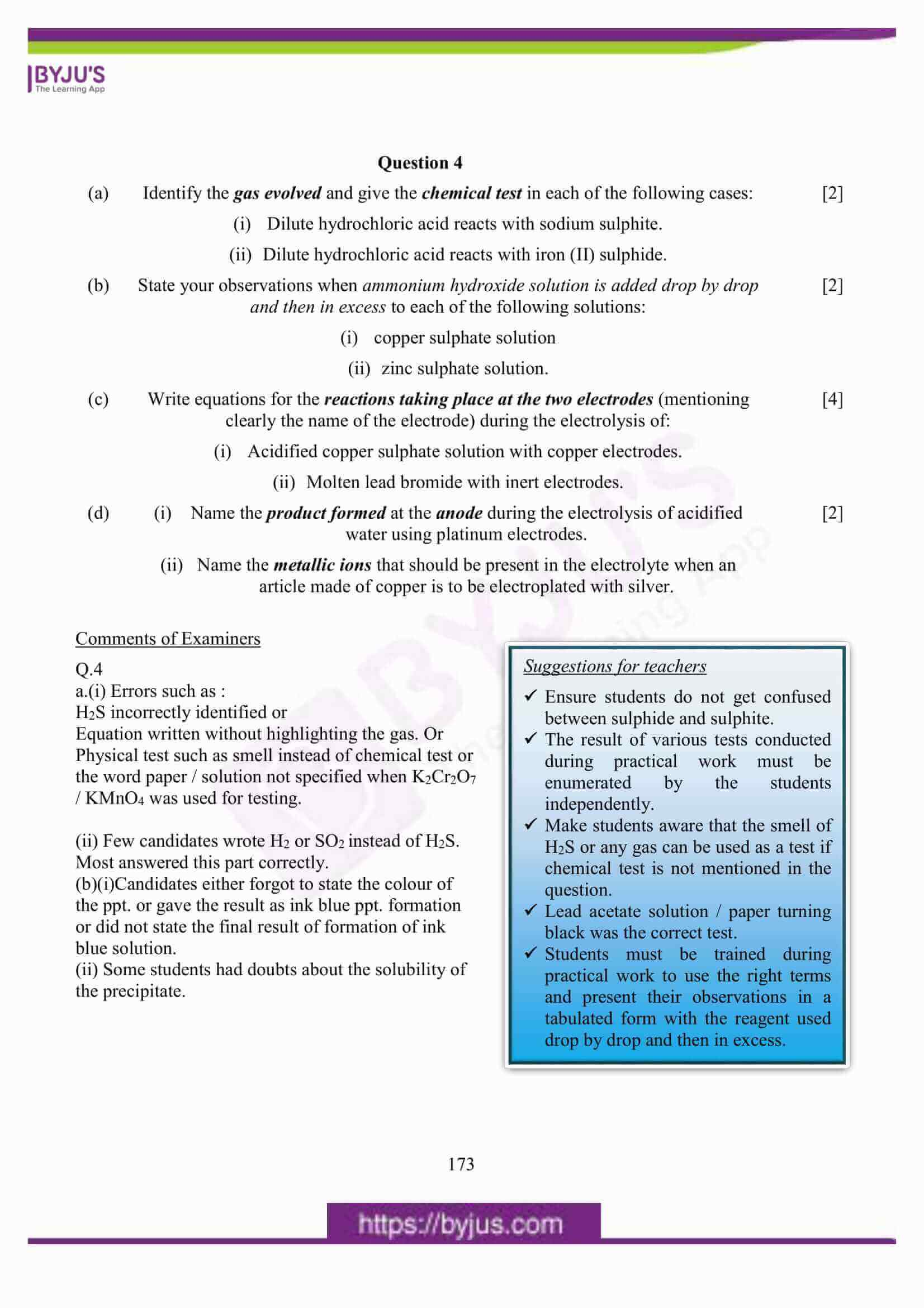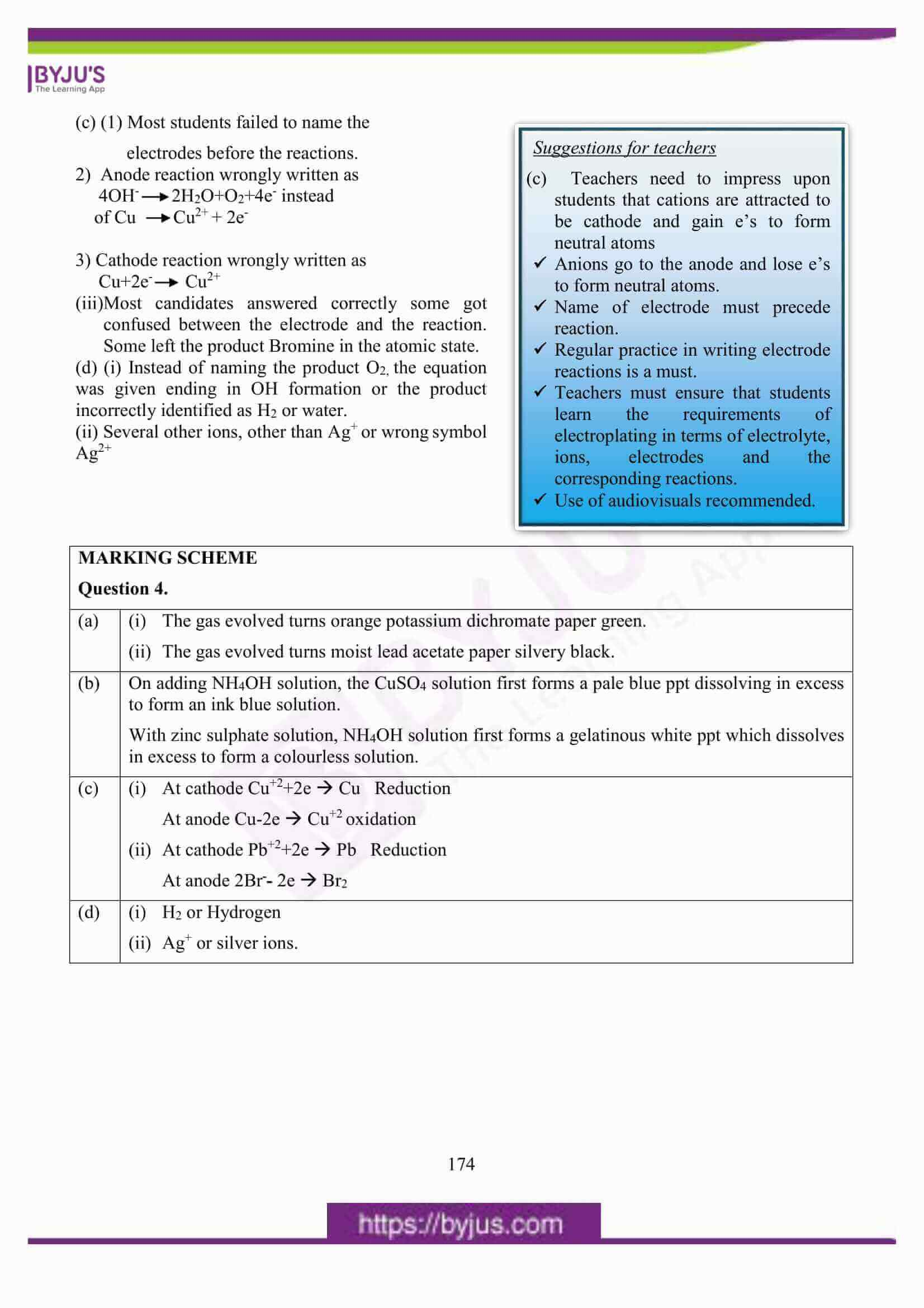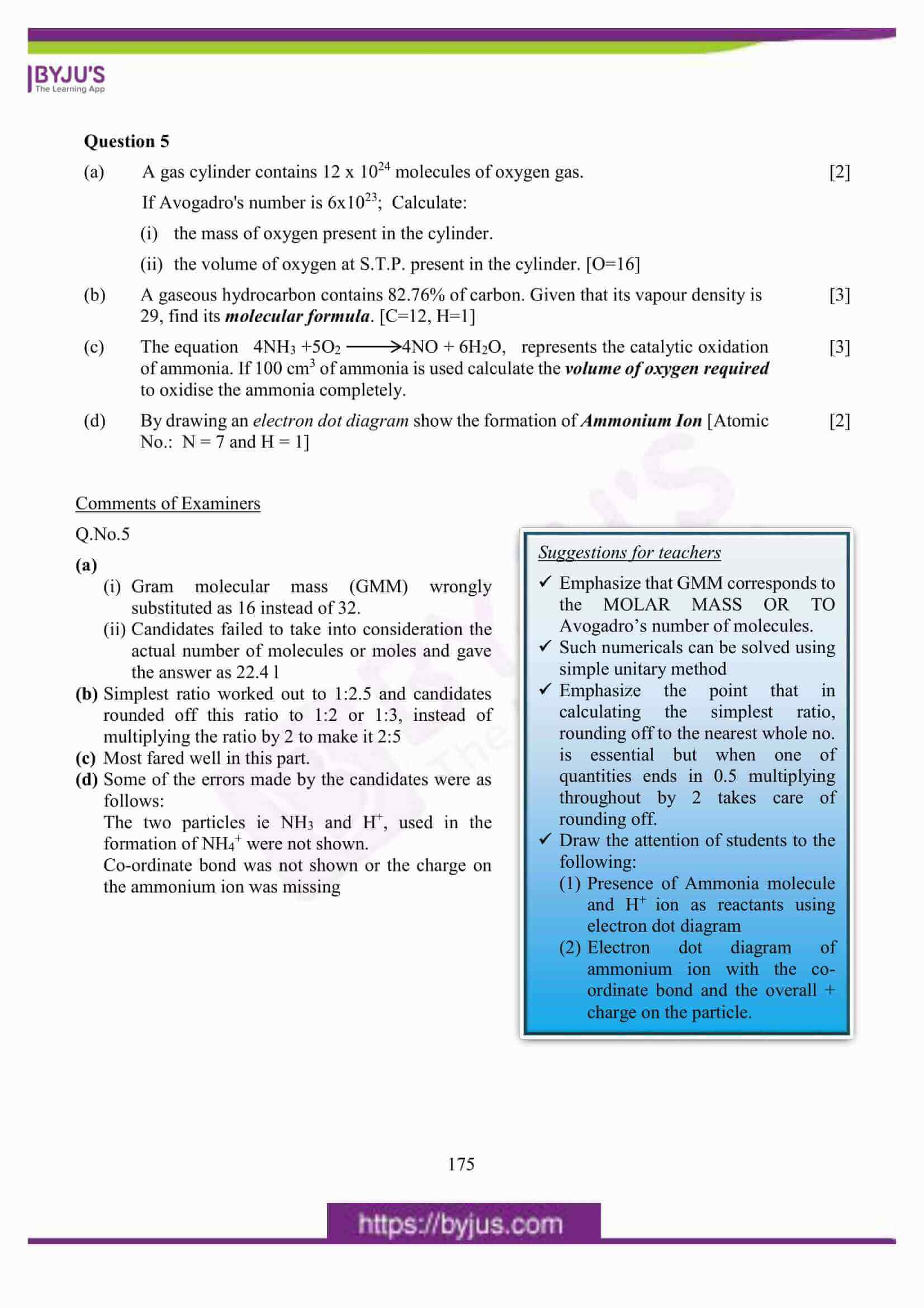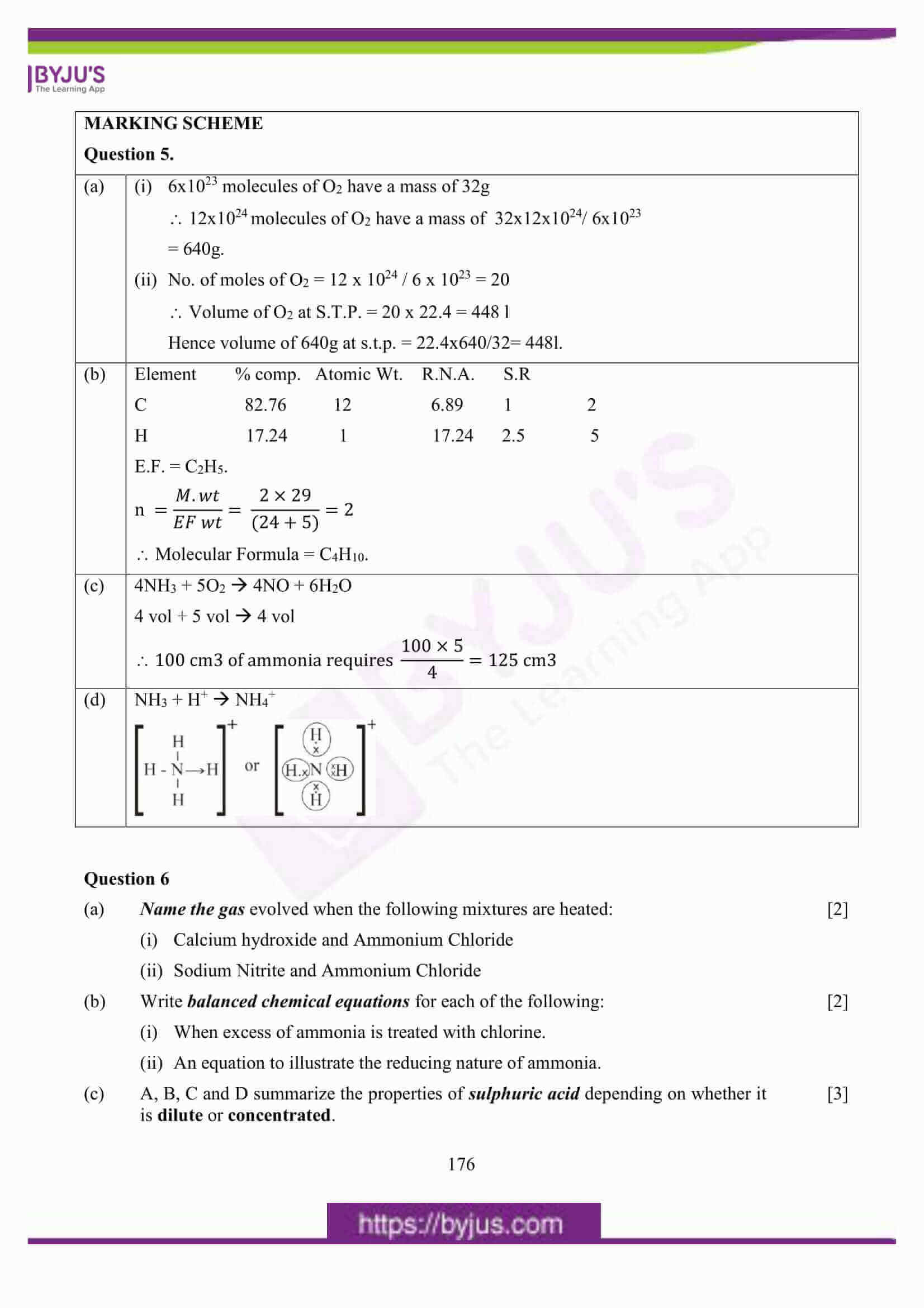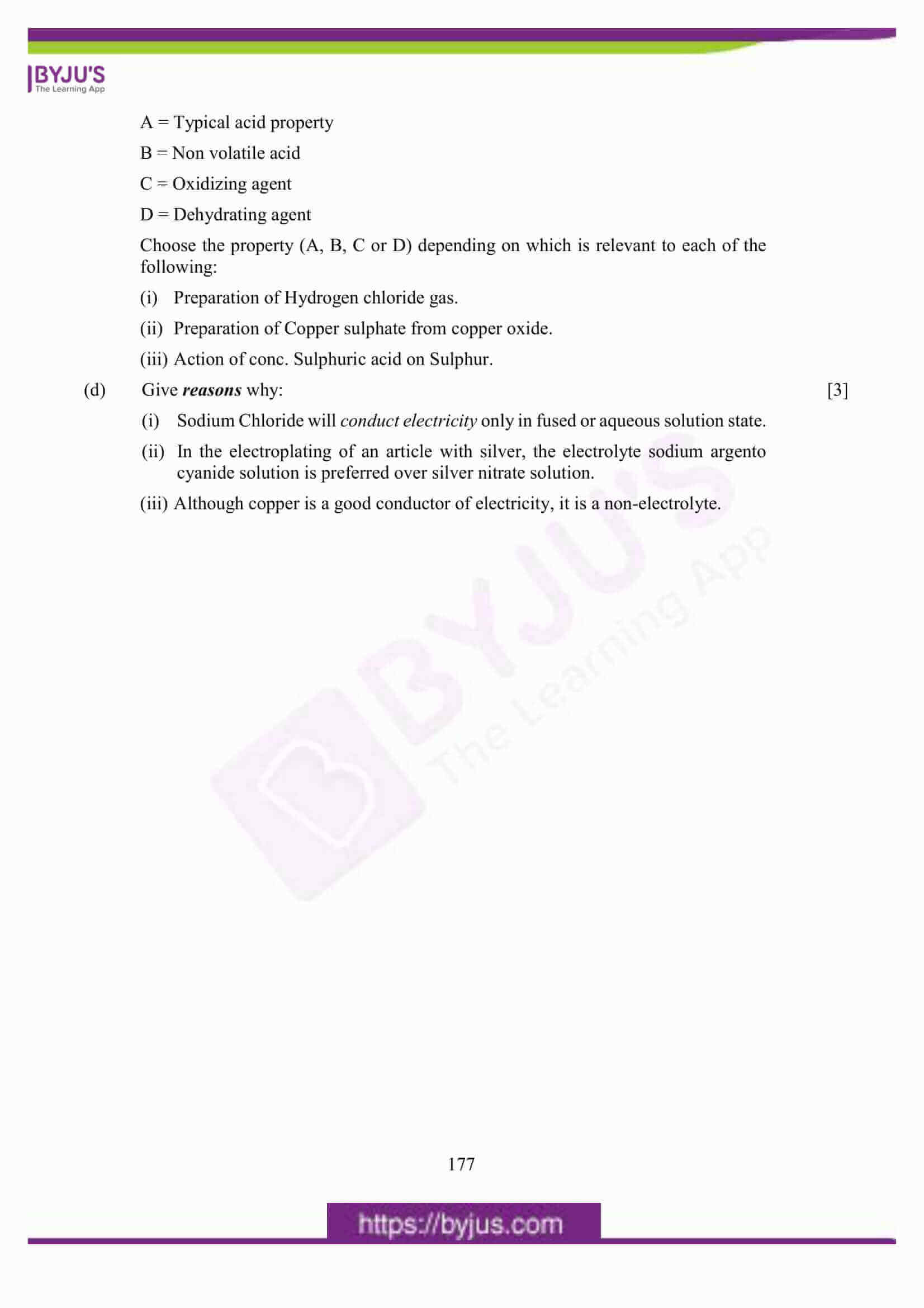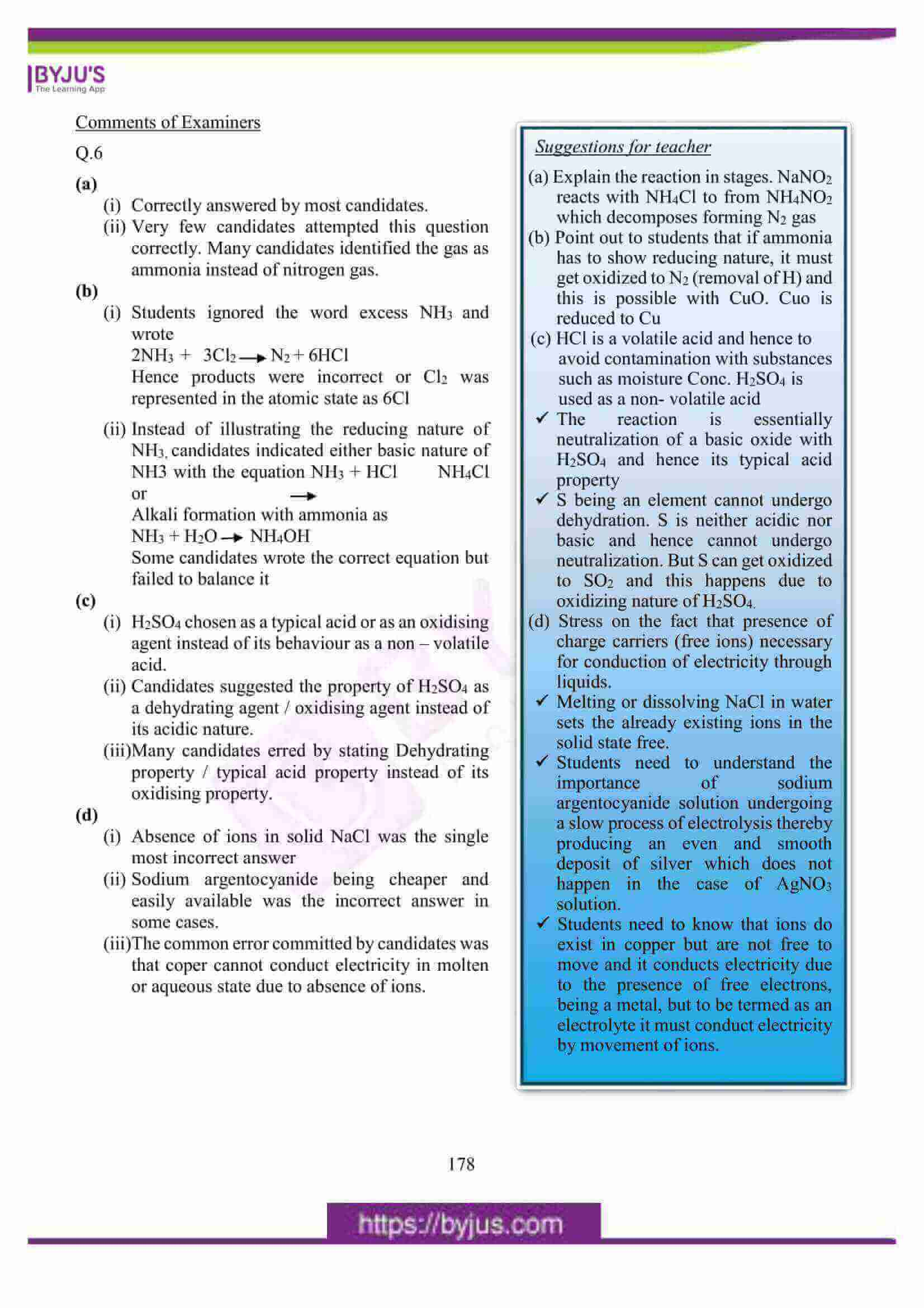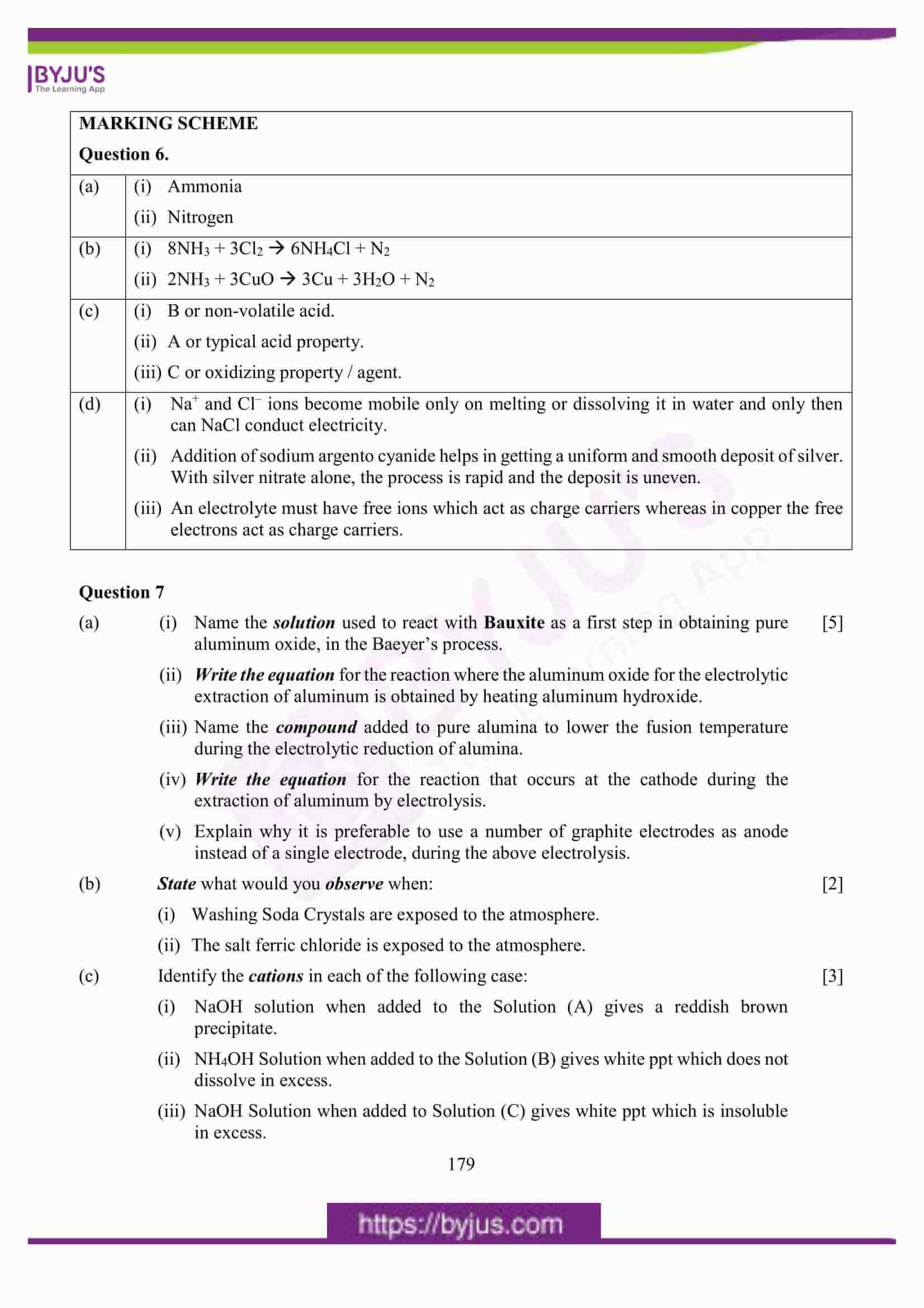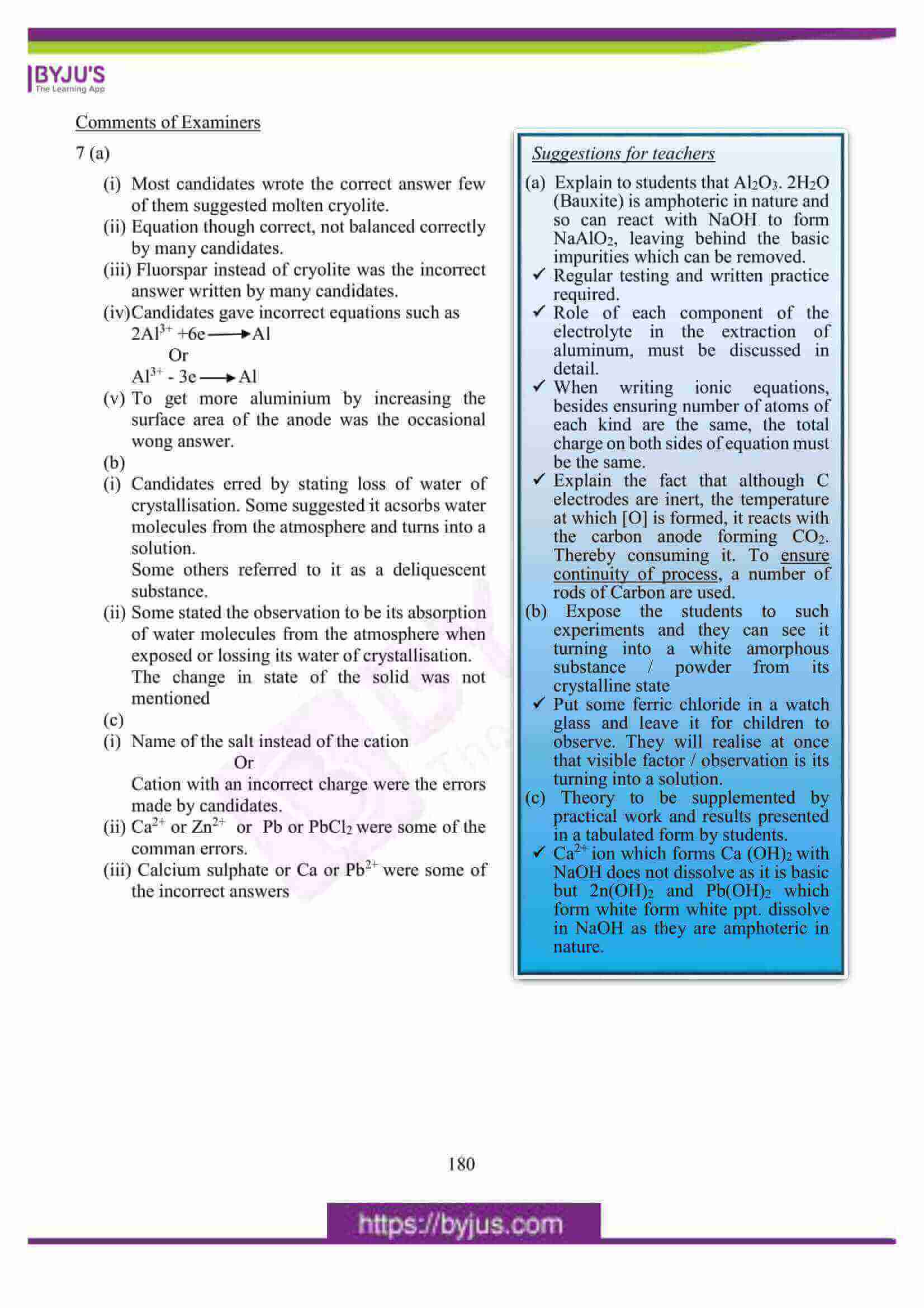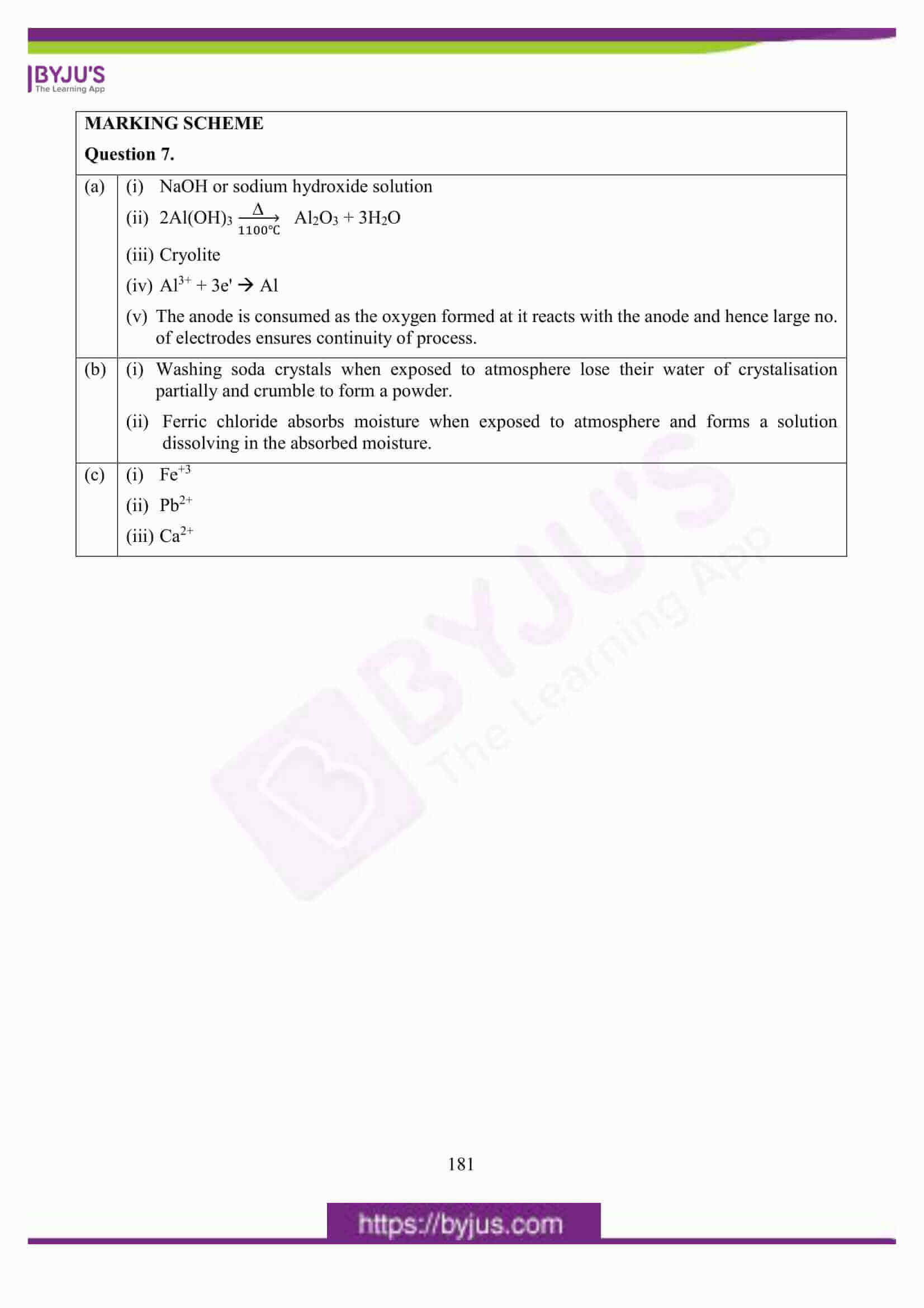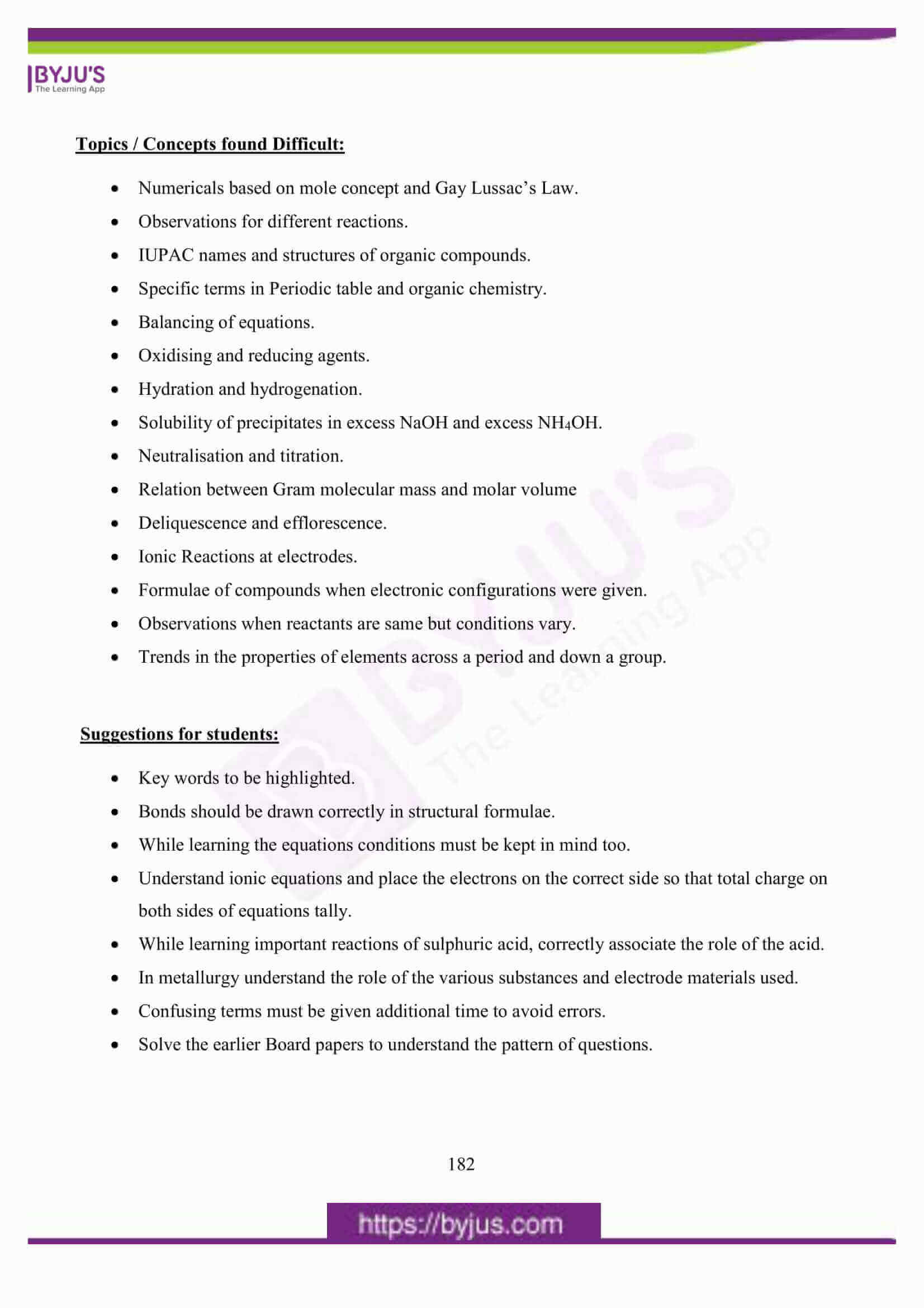## ICSE Class 10 Chemistry (Science Paper 2) Question Paper 2016 With Solution

### Question 1:

(a) Fill in the blanks with the choices given in brackets.

(i) Metals are good __________ (oxidizing agents / reducing agents) because they are electron __________ (acceptors/ donors).

(ii) Electrovalent compounds have __________ (high / low) melting points.

(iii) Higher the pH value of a solution, the more __________ (acidic / alkaline) it is.

(iv) __________ (AgCl / PbCl2), a white precipitate is soluble in excess NH4OH.

(v) Conversion of ethene to ethane is an example of __________ (hydration / hydrogenation).

(b) Choose the correct answer from the options given below:

(i) An element with the atomic number 19 will most likely combine chemically with the element whose atomic number is:

1. 17
2. 11
3. 18
4. 20

(ii) The ratio between the number of molecules in 2g of hydrogen and 32g

of oxygen is:

1. 1:2
2. 1:0.01
3. 1:1
4. 0.01:1
[Given that H=1, O=16]

(iii) The two main metals in Bronze are:

1. Copper and zinc
3. Copper and nickel
4. Copper and tin

(iv) The particles present in strong electrolytes are:

1. only molecules
2. mainly ions
3. ions and molecules
4. only atoms.

(v) The aim of the Fountain Experiment is to prove that:

1. HCl turns blue litmus red
2. HCl is denser than air
3. HCl is highly soluble in water
4. HCl fumes in moist air.

(c) Write balanced chemical equations for each of the following:

(i) Action of warm water on AlN.

(ii) Action of hot and concentrated Nitric acid on copper.

(iii) Action of Hydrochloric acid on sodium bicarbonate.

(iv) Action of dilute Sulphuric acid on Sodium Sulphite.

(v) Preparation of ethanol from Ethyl Chloride.

(i) Dilute Hydrochloric acid is added to Lead nitrate solution and the mixture is heated.

(ii) Barium chloride solution is mixed with Sodium Sulphate Solution.

(iii) Concentrated Sulphuric acid is added to Sugar Crystals.

(iv) Dilute Hydrochloric acid is added to Copper carbonate.

(v) Dilute Hydrochloric acid is added to Sodium thiosulphate.

(e) Identify the term/substance in each of the following:

(i) The tendency of an atom to attract electrons to itself when combined in a compound.

(ii) The method used to separate ore from gangue by preferential wetting.

(iii) The catalyst used in the conversion of ethyne to ethane.

(iv) The type of reactions alkenes undergo.

(v) The electrons present in the outermost shell of an atom.

(f) (i) A gas of mass 32gms has a volume of 20 litres at S.T.P. Calculate the gram molecular weight of the gas.

(ii) How much Calcium oxide is formed when 82g of calcium nitrate is heated? Also find the volume of nitrogen dioxide evolved:

2Ca(NO3)2 → 2CaO + 4NO2 + O2

(Ca = 40, N = 14, O = 16)

(g) Match the salts given in Column I with their method of preparation given in Column II:

 Column I Column II (i) Pb(NO3)2 from PbO A) Simple displacement (ii) MgCl2 from Mg B) Titration (iii) FeCl3 from Fe C) Neutralization (iv) NaNO3 from NaOH D) Precipitation (v) ZnCO3 from ZnSO4 E) Combination

(h) (i) Write the IUPAC names of each of the following: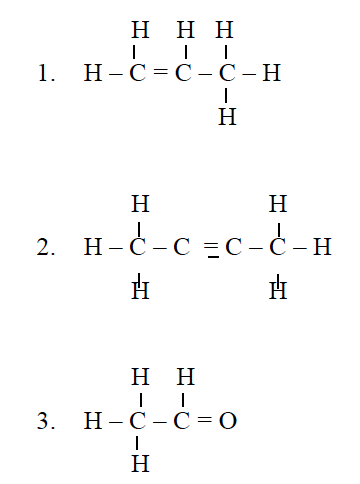(ii) Rewrite the following sentences by using the correct symbol > (greater than) or < (less than) in the blanks given:

1. The ionization potential of Potassium is _____ that of Sodium.
2. The electronegativity of Iodine is _____ that of Chlorine.

(a) (i) Reducing agents, donors

(ii) high

(iii) alkaline

(iv) AgCl

(v) hydrogenation

(b) (i) A or 17

(ii) C or 1:1

(iii) D or Cu & tin

(iv) B or mainly ions

(v) C or HCl is highly soluble in water.

(c) (i) AlN + 3H2O → Al (OH)3 + NH3

(ii) Cu + 4HNO3 → Cu(NO3)2 + 2H2O + 2NO2

(iii) NaHCO3 + HCl → NaCl + H2O + CO2

(iv) Na2SO3 + H2SO4 → Na2SO4 + H2O+ SO2

(v) C2H5Cl + KOH(aq) → C2H5OH + KCl

(d) (i) A white precipitate soluble on heating.

(ii) A white ppt is formed which is insoluble in all the mineral acids

(iii) Sugar chars to give black porous mass

(iv) A colourless and odourless gas evolves with brisk effervescence which turns lime water milky.

(v) Gas evolved which turns potassium dichromate paper from orange to green and yellow particles of Sulphur.

(e) (i) Electronegativity

(ii) Froth floatation

(iii) Nickel

(v) Valence electrons

(f) (i)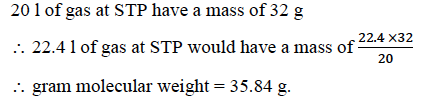(ii) 2Ca(NO3)2 → 2CaO + 4NO2 + O2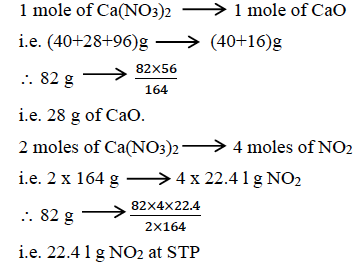(g) (i) C or Neutralization

(ii) A or Simple displacement

(iii) E or synthesis

(iv) B or Titration

(v) D or Precipitation

(h) (i)

1. Propene
2. but-2-yne
3. ethanal

(ii)

1. < or less than
2. < or less than

### Question 2:

(a) Use the letters only written in the Periodic Table given below to answer the questions that follow: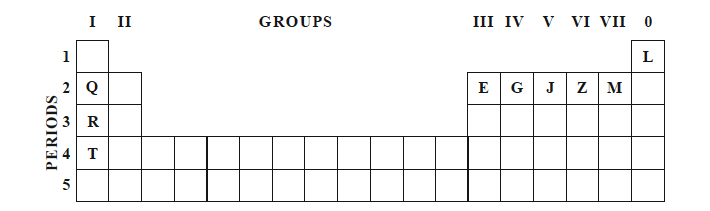(i) State the number of valence electrons in atom J.

(ii) Which element shown forms ions with a single negative charge?

(iii) Which metallic element is more reactive than R?

(iv) Which element has its electrons arranged in four shells?

(b) Fill in the blanks by selecting the correct word from the brackets:

(i) If an element has a low ionization energy then it is likely to be __________ (metallic / non metallic).

(ii) If an element has seven electrons in its outermost shell then it is likely to have the __________ (largest / smallest) atomic size among all the elements in the same period.

(c) The following table shows the electronic configuration of the elements W, X, Y, Z:

 Element W X Y Z Electronic Configurations 2,8,1 2,8,7 2,5 1

Answer the following questions based on the table above:

(i) What type of Bond is formed between:

1. W and X
2. Y and Z

(ii) What is the formula of the compound formed between :

1. X and Z
2. W and X

(a) (i) five

(ii) M

(iii) T

(iv) T

(b) (i) metallic

(ii) smallest

(c) (i)

1. electrovalent bond or Ionic Bond
2. Covalent Bond

(ii)

1. ZX
2. WX

### Question 3:

(a) Write a balanced chemical equation for each of the following:

(i) Burning of ethane in plentiful supply of air.

(ii) Action of water on Calcium carbide.

(iii) Heating of Ethanol at 170oC in the presence of conc. Sulphuric acid.

(b) Give the structural formulae of each of the following

(i) 2-methyl propane

(ii) Ethanoic acid

(iii) Butan – 2 – ol

(c) Equation for the reaction when compound A is bubbled through bromine dissolved

in carbon tetrachloride is as follows: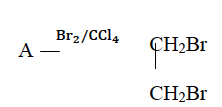(i) Draw the structure of A.

(ii) State your observation during this reaction.

(d) Fill in the blanks using the appropriate words given below:

(Sulphur dioxide, Nitrogen dioxide, Nitric oxide, Sulphuric acid)

(i) Cold, dilute nitric acid reacts with copper to give __________.

(ii) Hot, concentrated nitric acid reacts with sulphur to form __________.

(a)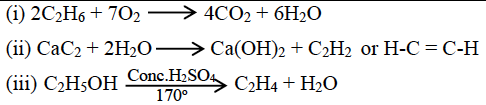(b)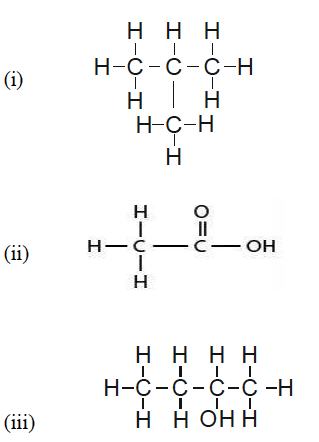(c)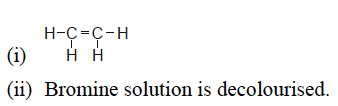(d) (i) Nitric oxide

(iii) Sulphuric acid

### Question 4:

(a) Identify the gas evolved and give the chemical test in each of the following cases:

(i) Dilute hydrochloric acid reacts with sodium sulphite.

(ii) Dilute hydrochloric acid reacts with iron (II) sulphide.

(b) State your observations when ammonium hydroxide solution is added drop by drop and then in excess to each of the following solutions:

(i) copper sulphate solution

(ii) zinc sulphate solution.

(c) Write equations for the reactions taking place at the two electrodes (mentioning clearly the name of the electrode) during the electrolysis of:

(i) Acidified copper sulphate solution with copper electrodes.

(ii) Molten lead bromide with inert electrodes.

(d) (i) Name the product formed at the anode during the electrolysis of acidified water using platinum electrodes.

(ii) Name the metallic ions that should be present in the electrolyte when an article made of copper is to be electroplated with silver.

(a) (i) The gas evolved turns orange potassium dichromate paper green.

(ii) The gas evolved turns moist lead acetate paper silvery black.

(b) On adding NH4OH solution, the CuSO4 solution first forms a pale blue ppt dissolving in excess to form an ink blue solution.

With zinc sulphate solution, NH4OH solution first forms a gelatinous white ppt which dissolves in excess to form a colourless solution.

(c)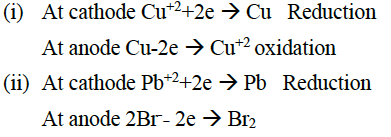(d) (i) H2 or Hydrogen

(ii) Ag+ or silver ions.

### Question 5:

(a) A gas cylinder contains 12 x 1024 molecules of oxygen gas.

If Avogadro’s number is 6×1023; Calculate:

(i) the mass of oxygen present in the cylinder.

(ii) the volume of oxygen at S.T.P. present in the cylinder. [O=16]

(b) A gaseous hydrocarbon contains 82.76% of carbon. Given that its vapour density is 29, find its molecular formula. [C=12, H=1]

(c) The equation 4NH3 + 5O2 → 4NO + 6H2O, represents the catalytic oxidation of ammonia. If 100 cm3 of ammonia is used calculate the volume of oxygen required to oxidise the ammonia completely.

(d) By drawing an electron dot diagram show the formation of Ammonium Ion [Atomic No.: N = 7 and H = 1]

(a)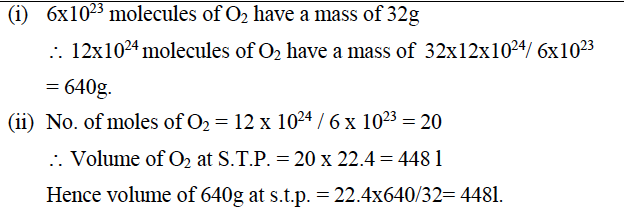(b)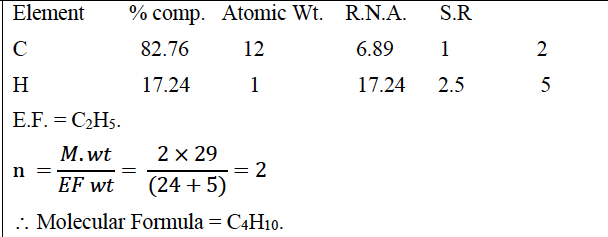(c)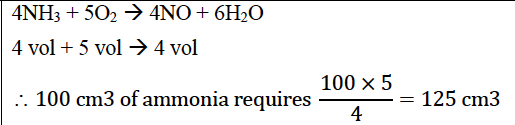(d)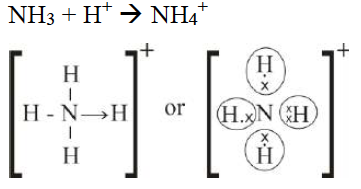### Question 6:

(a) Name the gas evolved when the following mixtures are heated:

(i) Calcium hydroxide and Ammonium Chloride

(ii) Sodium Nitrite and Ammonium Chloride

(b) Write balanced chemical equations for each of the following:

(i) When excess of ammonia is treated with chlorine.

(ii) An equation to illustrate the reducing nature of ammonia.

(c) A, B, C and D summarize the properties of sulphuric acid depending on whether it is dilute or concentrated.

A = Typical acid property

B = Non volatile acid

C = Oxidizing agent

D = Dehydrating agent

Choose the property (A, B, C or D) depending on which is relevant to each of the following:

(i) Preparation of Hydrogen chloride gas.

(ii) Preparation of Copper sulphate from copper oxide.

(iii) Action of conc. Sulphuric acid on Sulphur.

(d) Give reasons why:

(i) Sodium Chloride will conduct electricity only in fused or aqueous solution state.

(ii) In the electroplating of an article with silver, the electrolyte sodium argento cyanide solution is preferred over silver nitrate solution.

(iii) Although copper is a good conductor of electricity, it is a non-electrolyte.

(a) (i) Ammonia

(ii) Nitrogen

(b) (i) 8NH3 + 3Cl2 → 6NH4Cl + N2

(ii) 2NH3 + 3CuO → 3Cu + 3H2O + N2

(c) (i) B or non-volatile acid.

(ii) A or typical acid property.

(iii) C or oxidizing property / agent.

(d) (i) Na+ and Cl ions become mobile only on melting or dissolving it in water and only then can NaCl conduct electricity.

(ii) Addition of sodium argento cyanide helps in getting a uniform and smooth deposit of silver.

With silver nitrate alone, the process is rapid and the deposit is uneven.

(iii) An electrolyte must have free ions which act as charge carriers whereas in copper the free

electrons act as charge carriers.

### Question 7:

(a) (i) Name the solution used to react with Bauxite as a first step in obtaining pure aluminum oxide, in the Baeyer’s process.

(ii) Write the equation for the reaction where the aluminum oxide for the electrolytic extraction of aluminum is obtained by heating aluminum hydroxide.

(iii) Name the compound added to pure alumina to lower the fusion temperature during the electrolytic reduction of alumina.

(iv) Write the equation for the reaction that occurs at the cathode during the extraction of aluminum by electrolysis.

(v) Explain why it is preferable to use a number of graphite electrodes as anode instead of a single electrode, during the above electrolysis.

(b) State what would you observe when:

(i) Washing Soda Crystals are exposed to the atmosphere.

(ii) The salt ferric chloride is exposed to the atmosphere.

(c) Identify the cations in each of the following case:

(i) NaOH solution when added to the Solution (A) gives a reddish brown

precipitate.

(ii) NH4OH Solution when added to the Solution (B) gives white ppt which does not dissolve in excess.

(iii) NaOH Solution when added to Solution (C) gives white ppt which is insoluble in excess.

(a) (i) NaOH or sodium hydroxide solution

(ii)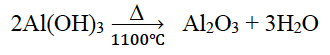(iii) Cryolite

(iv) Al3+ + 3e’ → Al

(v) The anode is consumed as the oxygen formed at it reacts with the anode and hence large no. of electrodes ensures continuity of process.

(b) (i) Washing soda crystals when exposed to atmosphere lose their water of crystalisation partially and crumble to form a powder.

(ii) Ferric chloride absorbs moisture when exposed to atmosphere and forms a solution dissolving in the absorbed moisture.

(c) (i) Fe+3

(ii) Pb2+

(iii) Ca2+

We hope ICSE Class 10 Chemistry Question Paper Solution 2016 must have provided an insight into the 2016 Question paper and their solutions. Going through this paper solution will surely help students in their exam preparation. Students can also find the answers of other papers of ICSE Class 10 Previous Years Questions by clicking here. Happy Learning and stay tuned to BYJU’S for the latest update on ICSE/CBSE/State Boards/Competitive exams. Also, don’t forget to download the BYJU’S App.